[Introduction]  [Signal arithmetic]  [Signals and noise]   [Smoothing]   [Differentiation]  [Peak Sharpening]  [Harmonic analysis]   [Fourier convolution]  [Fourier deconvolution]  [Fourier filter]  [Wavelets]   [Peak area measurement]  [Linear Least Squares]  [Multicomponent Spectroscopy]  [Iterative Curve Fitting]  [Hyperlinear quantitative absorption spectrophotometry] [Appendix and Case Studies]  [Peak Finding and Measurement]  [iPeak]   [iSignal]  [Peak Fitters]   [iFilter]  [iPower]  [List of downloadable software]  [Interactive tools]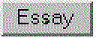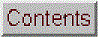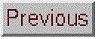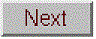## Peak Finding and Measurement

### [findpeaksx]  [findpeaksG and related]  [findvalleys]  [Background correction]  [Signal-to-noise ratio]  [Peak Start and End]  [Finding and fitting peaks]  [Comparison of peak finding functions]  [Using the peak table]  [Peak Identification]  [iPeak]  [iPeak demo functions]   [Spreadsheets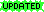]  [Animated Demonstration]  [Have a question? Email me]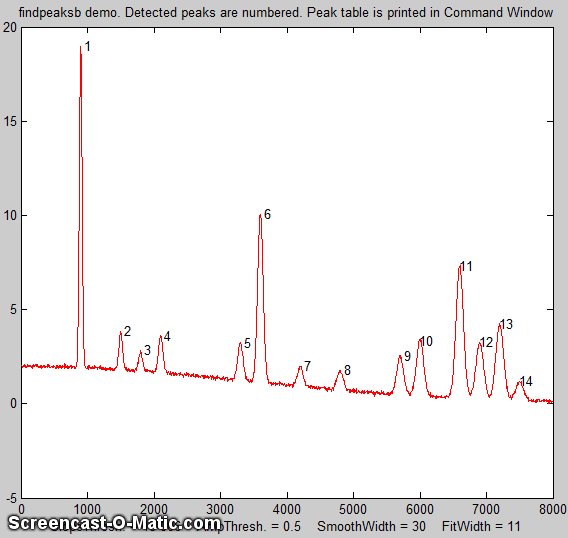A common requirement in scientific data processing is to detect peaks in a signal and to measure their positions, heights, widths, and/or areas. One way to do this is to make use of the fact that the first derivative of a peak has a downward-going zero-crossing at the peak maximum. But the presence of random noise in real experimental signal will cause many false zero-crossing simply due to the noise. To avoid this problem, the technique described here first smooths the first derivative of the signal, before looking for downward-going zero-crossings, and then it takes only those zero crossings whose slope exceeds a certain predetermined minimum (called the "slope threshold") at a point where the original signal exceeds a certain minimum (called the "amplitude threshold"). By carefully adjusting the smooth width, slope threshold, and amplitude threshold, it's possible to detect only the desired peaks and ignore peaks that are too small, too wide, or too narrow. Moreover, this technique can be extended to estimate the position, height, and width of each peak by least-squares curve-fitting of a segment of the original unsmoothed signal in the vicinity of the zero-crossing. Thus, even if heavy smoothing of the first derivative is necessary to provide reliable discrimination against noise peaks, the peak parameters extracted by curve fitting are not distorted by the smoothing, and the effect of random noise in the signal is reduced by curve fitting over multiple data points in the peak. This technique is capable of measuring peak positions and heights quite accurately, but the measurements of peak widths and areas is most accurate if the peaks are Gaussian in shape (or Lorentzian, in the variant findpeaksL). For the most accurate measurement of other peak shapes, or of highly overlapped peaks, or of peak superimposed on a baseline, the related functions findpeaksb.m, findpeaksb3.m, findpeaksfit.m utilize non-linear iterative curve fitting with selectable peak shape models and baseline correction modes.

The routine is now available in several different versions which are described below:

(1) a set of command-line functions for Matlab or Octave, each linked to its description: findpeaksx, findpeaksxw, findpeaksG, findvalleys, findpeaksL, measurepeaks, findpeaksG2d, findpeaksb, findpeaksb3, findpeaksplot, findpeaksplotL, peakstats, findpeaksE, findpeaksGSS, findpeaksLSS, findpeaksT, findpeaksfit, autofindpeaks and autopeaks. These can be used as components in creating your own custom scripts and functions. Don't confuse with the "findpeaks" function in Matlab's Signal Processing Toolbox; that's a completely different algorithm.

(2) an interactive keypress-operated function, called iPeak (ipeak.m), for adjusting the peak detection criteria interactively to optimize for any particular peak type (Matlab only). iPeak runs in the Figure window and use a simple set of keystroke commands to reduce screen clutter, minimize overhead, and maximize processing speed.

(3) A set of spreadsheets, available in Excel and in OpenOffice formats.

(4)
Real-time peak detection in Matlab is discussed in Appendix Y.

Click here to download the ZIP file "PeakFinder.zip", which includes findpeaksG.m and its variants, ipeak.m, and a sample data file and demo scripts for testing. You can also download iPeak and other programs of mine from the Matlab File Exchange.

#### Simple fast functions for detecting peaks in smooth or previously smoothed data.

allpeaks(x,y) A super-simple peak detector for x,y, data sets that lists every y value that has lower y values on both sides; allvalleys.m is the same for valleys, lists every y value that has higher y values on both sides.

peaksat.m. (Peaks Above Threshold) Syntax: P=peaksat(x,y,threshold). This function detects every y value that (a) has lower y values on both sides and (b) is above the specified threshold. Returns a 2 by n matrix P with the x and y values of each peak, where n is the number of detected peaks.

###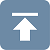P=findpeaksx(x, y, SlopeThreshold, AmpThreshold, SmoothWidth, PeakGroup, smoothtype)
P=findpeaksxw(x, y, SlopeThreshold, AmpThreshold, SmoothWidth, PeakGroup, smoothtype)
Simple and fast command-line functions to locate and count the positive peaks in a noisy data sets. Detects peaks by looking for downward zero-crossings in the smoothed first derivative that exceed SlopeThreshold and peak amplitudes that exceed AmpThreshold, and returns a list (in matrix P) containing the peak number and the measured position and height of each peak (and for the variant findpeaksxw, the full width at half maximum, determined by calling the halfwidth.m function) .
It can find and count over 10,000 peaks per second in very large signals. The data are passed to the findpeaksx function in the vectors x and y (x = independent variable, y = dependent variable). The other parameters are user-adjustable:
SlopeThreshold - Slope of the smoothed first-derivative that is taken to indicate a peak. This discriminates on the basis of peak width. Larger values of this parameter will neglect broad features of the signal. A reasonable initial value for Gaussian peaks is 0.7*WidthPoints^-2, where WidthPoints is the number of data points in the half-width (FWHM) of the peak.
AmpThreshold - Discriminates on the basis of peak height. Any peaks with height less than this value are ignored.
SmoothWidth - Width of the smooth function that is applied to data before the slope is measured. Larger values of SmoothWidth will neglect small, sharp features. A reasonable value is typically about equal to 1/2 of the number of data points in the half-width of the peaks.
PeakGroup - The number of points around the "top part" of the (unsmoothed) peak that are taken to estimate the peak heights. If the value of PeakGroup is 1 or 2, the maximum y value of the 1 or 2 points at the point of zero-crossing is taken as the peak height value; if PeakGroup is n is 3 or greater, the average of the next n points is taken as the peak height value. For spikes or very narrow peaks, keep PeakGroup=1 or 2; for broad or noisy peaks, make PeakGroup larger to reduce the effect of noise.

Smoothtype determines the smoothing algorithm (see http://terpconnect.umd.edu/~toh/spectrum/Smoothing.html)
If smoothtype=1, rectangular (sliding-average or boxcar)
If smoothtype=2, triangular (2 passes of sliding-average)
If smoothtype=3, pseudo-Gaussian (3 passes of sliding-average)
Basically, higher values yield  greater reduction in high-frequency noise, at the expense of slower execution. For a comparison of these smoothing types, see SmoothingComparison.html.

The demonstration scripts demofindpeaksx.m and demofindpeaksxw.m finds, numbers, plots, and measures noisy peaks with unknown random positions. (Note that if two peaks overlap too much, the reported width will be the width of the blended peak, in which cases it's better to use findpeaksG.m, below).

Speed demonstration; detecting 12,000 peaks (in Matlab, on a typical desktop PC):

>> x=[0:.01:500]'; y=x.*sin(x.^2).^2; tic; P=peaksat(x,y,0); toc; NumPeaks=length(P)
Elapsed time is 0.047 seconds in Matlab 2019 for NumPeaks = 12028, which is 2.5532e+05 peaks per second.

>> x=[0:.01:500]'; y=x.*sin(x.^2).^2; tic; P=findpeaksx(x,y,0,0,3,3); toc; NumPeaks=length(P)
Elapsed time is 0.33 seconds in Matlab 2019 for NumPeaks = 12028, which is 36,000 peaks per second.

####### Functions for detecting and measuring Gaussian peaks, findpeaksG.m, or Lorentzian peaks, findpeaksL.m, for Matlab or Octave.

P=findpeaksG(x, y, SlopeThreshold, AmpThreshold, SmoothWidth, FitWidth, smoothtype)
P=findpeaksL(x, y, SlopeThreshold, AmpThreshold, SmoothWidth, FitWidth, smoothtype)
These functions locate the positive peaks in a noisy data set, performs a least-squares curve-fit of a Gaussian or Lorentzian function to the top part of the peak, and computes the position, height, and width (FWHM) of each peak from that least-squares fit. (The 6th input argument, FitWidth, is the number of data points around each peak top that is fit). The other arguments are that same as findpeaksx. Returns a list (in matrix P) containing the peak number and the estimated position, height, width, and area of each peak. It can find and curve-fit over 2800 peaks per second in very large signals.
(This is useful primarily for signals that have several data points in each peak, not for spikes that have only one or two points, for which findpeaksx is better).

>> x=[0:.01:50]; y=(1+cos(x)).^2; P=findpeaksG(x,y,0,-1,5,5); plot(x,y)
P =
1       6.2832       4       2.3548       10.028
2       12.566       4       2.3548       10.028
3        18.85       4       2.3548       10.028...
...

The function findpeaksplot.m is a simple variant of findpeaksG.m that also plots the x,y data and numbers the peaks on the graph (if any are found). The function findpeaksplotL.m does the same thing optimized for Lorentzian peak.

findpeaksSG.m is a segmented variant of the findpeaksG function, with the same syntax, except that the four peak detection parameters can be vectors, dividing up the signal into regions that are optimized for peaks of different widths. Any number of segments can be declared, based on the length of the third (SlopeThreshold) input argument.
(Note: you only need to enter vectors for those parameters that you want to vary between segments; to allow any of the other peak detection parameters to remain unchanged across all segments, simply enter a single scalar value for that parameter; only the SlopeThreshold must be a vector). The following example declares two segments, with AmpThreshold remaining the same in both segments:

SlopeThreshold=[0.001 .0001]
AmpThreshold=.2;
SmoothWidth=[5 10];
FitWidth=[10 20];
P=findpeaksSG(x, y,
SlopeThreshold, AmpThreshold, SmoothWidth, FitWidth,3)

FindpeaksSL.m is the same thing for Lorentzian peaks.

A related function is findpeaksSGw.m which is similar to the above except that is uses wavelet denoising instead of regular smoothing. It takes the wavelet level rather than the smooth width as an input argument. The script TestPrecisionFindpeaksSGvsW.m compares the precision and accuracy for peak position and height measurement for both the findpeaksSG.m and findpeaksSGw.m functions, finding that there is little to be gained in most cases by using the wavelet denoise instead of smoothing. That is mainly because in either case the peak parameter measurements are based on least-squares fitting to the raw, not the smoothed, data at each detected peak location, so the usual wavelet denoising advantage of avoiding smoothing distortion does not apply here.

In the graphic example shown on the right, the demonstration script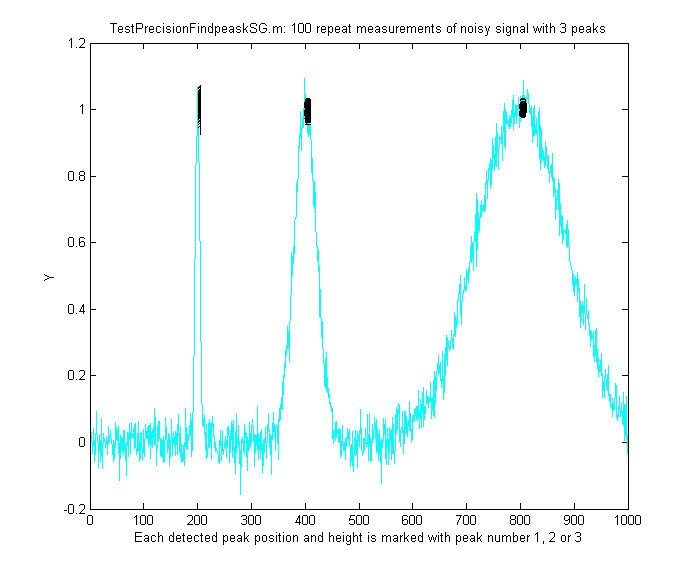TestPrecisionFindpeaksSG.m creates a noisy signal with three peaks of widely different widths, detects and measures the peak positions, heights and widths of each peak using findpeaksSG, then prints out the percent relative standard deviations of parameters of the three peaks in 100 measurements with independent random noise. With 3-segment peak detection parameters, findpeaksSG reliably detects and accurately measures all three peaks. In contrast, findpeaksG, tuned to the middle peak (using line 26 instead of line 25), measures the first and last peaks poorly, because the peak detection parameters are far from optimum for those peak widths. You can also see that the precision of peak height measurements gets progressively better (smaller relative standard deviation) the larger the peak widths, simply because there are more data points in wider peaks. (You can change any of the variables in lines 10-18).

One difficulty with the above peak finding functions it is annoying to have to estimate the values of the peak detection parameters that you need to use for your signals. A quick way to estimate these is to use autofindpeaks.m, which is similar to findpeaksG.m except that you can optionally leave out the peak detection parameters and just write “autofindpeaks(x, y)” or “autofindpeaks(x, y, n)”, where n is the "peak capacity", roughly the number of peaks that would fit into that signal record (greater n looks for many narrow peaks; smaller n looks for fewer wider peaks and neglects the fine structure). Basically n allows you to quickly adjust all of the peaks detection parameters at once just by changing a single number. In addition, if you do leave out the explicit peak detection parameters, autofindpeaks will print out the numerical input argument list that it uses in the command window, so you can copy, paste, and edit for use with any of the findpeaks... functions. If you call autofindpeaks with the output arguments [P,A]=autofindpeaks(x,y,n), it returns the calculated peak detection parameters as a 4-element row vector A, which you can then pass on to other functions such as measurepeaks, effectively giving that function the ability to calculate the peak detection parameters from a single single number n. For example, a quick visual estimate of the following signal indicates about 20 peaks, so you use 20 as the third input argument:
x=[0:.1:50];
y=10+10.*sin(x).^2+randn(size(x));
[P,A]=autofindpeaks(x,y,20);

Then you can use the values of the vector A as the peak detection parameters for other peak detection functions, such as P=findpeaksG(x,y,A(1),A(2),A(3),A(4),1) or P=measurepeaks(x,y,A(1),A(2),A(3),A(4),1). You will probably want to fine-tune the amplitude threshold A(2) manually for your own needs.

Type "help autofindpeaks" and run the examples there. autofindpeaksplot.m is the same but also plots and numbers the peaks. The script testautofindpeaks.m runs all the examples in the help file, plots the data and numbers the peaks (like autofindpeaksplot.m), with a 1-second pause between each example (animated graphic).

Optimization of peak finding
Finding peaks in a signal depends on distinguishing between legitimate peaks and other feature like noise and baseline changes. Ideally, a peak detector should detect all the legitimate peaks and ignore all the other features. This requires that a peak detector be "tuned" or optimized for the desired peaks. For example, the Matlab/Octave demonstration script OnePeakOrTwo.m creates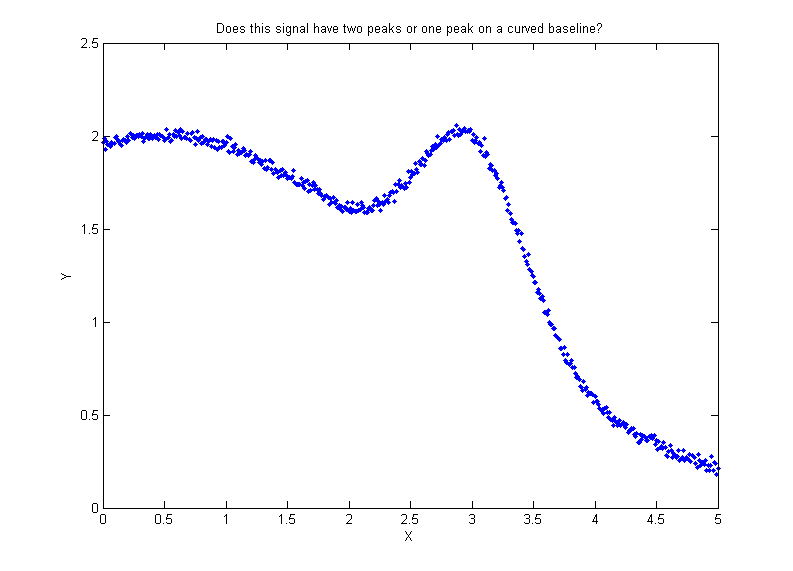a signal (shown on the right) that might be interpreted as either one peak at x=3 on a curved baseline or as two peaks at x=.5 and x=3, depending on context. The peak finding algorithms described here have input arguments that allow some latitude for adjustment. In this example script, the "SlopeThreshold" argument is adjusted to detect just one or both of those peaks.

Similarly, the signal shown in the figure on the left below could be interpreted as either as two broad noisy peaks or as 25 little narrow peaks on a two-humped background. The findpeaks... functions allow either interpretation, depending in the peak detection parameters. The optimum values of the input arguments for findpeaksG and related functions depend on the signal and on which features of the signal are important for your work. Rough values for these parameters can be estimated based on the width of the peaks that you wish to detect, as described above, but for the greatest control it will be best to fine-tune these parameters for your particular signal. A simple way to do that is to use autopeakfindplot(x, y, n) and adjust n until it finds the peak you want; it will print out the numerical input argument list so you can copy, paste, and edit for use with any of the findpeaks... functions. A more flexible way, if you are using Matlab, is to use the interactive peak detector iPeak (described below), which allows you to adjust all of these parameters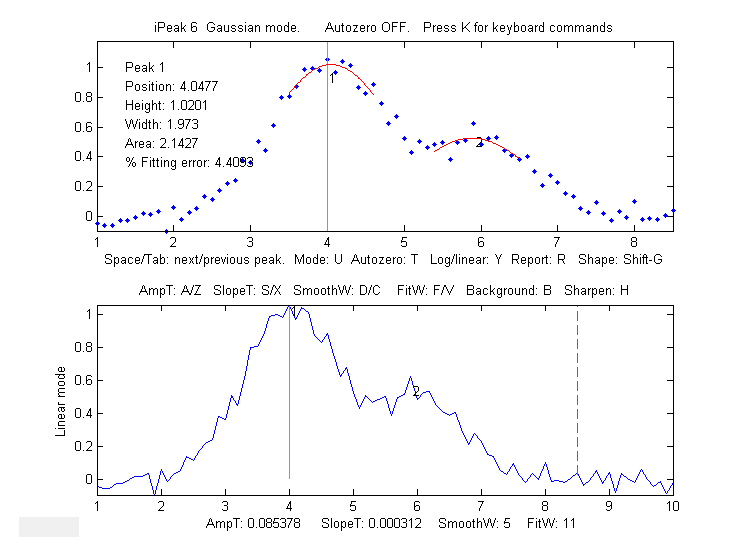individually by simple keypresses and displays the results graphically and instantly. The script FindpeaksComparison shows how findpeakG compares to the other peak detection functions when applied to a computer-generated signal with multiple peaks with variable types and amounts of baseline and random noise. By itself, autofindpeaks.m, findpeaksG and findpeaksL do not correct for a non-zero baseline; if your peaks are superimposed on a baseline, you should subtract the baseline first or use the other peak detection functions that do correct for the baseline.

In the example shown on the left (using the interactive peak detector iPeak program described below), suppose that the important parts of the signal are two broad peaks at x=4 and x=6, the second one half the height of the first. The small jagged features are just random noise. We want to detect the two peaks but ignore the noise. (The detected peaks are numbered 1,2,3,...in the lower panel of this graphic). This is what it looks like if the AmpThreshold is too small or too large, if the SlopeThreshold is too small or too large, if the SmoothWidth is too small or too large, if the FitWidth is too small or too large If these parameters are within the optimum range for this measurement objective, the findpeaksG functions will return something like this (although the exact values will vary with the noise and with the value of FitWidth):

Peak#    Position    Height     Width     Area
1      3.9649      0.99919    1.8237    1.94
2      5.8675      0.53817    1.6671    0.955

How is 'findpeaksG' different from 'max' in Matlab or 'findpeaks' in the Signal Processing Toolkit?
The 'max' function simply returns the largest single value in a vector.  Findpeaks in the Signal Processing Toolbox can be used to find the values and indices of all the peaks in a vector that are higher than a specified peak height and are separated from their neighbors by a specified minimum distance. My version of findpeaks (findpeaksG) accepts both an independent variable (x) and dependent variable (y) vectors, finds the places where the average curvature over a specified region is concave down, fits that region with a least-squares fit, and returns the peak position (in x units), height, width, and area, of any peak that exceeds a specified height. For example, let's create a noisy series of peaks (plotted on the right) a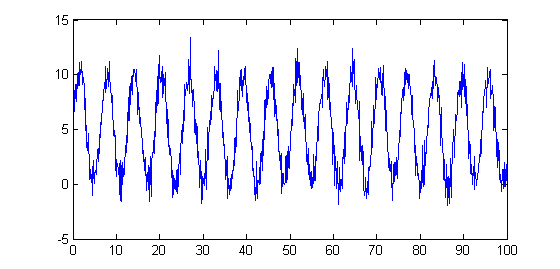nd apply both of these findpeaks functions to the resulting data.

x=[0:.1:100];
y=5+5.*sin(x)+randn(size(x));
plot(x,y)

Now, most people just looking at this plot of data would count 16 peaks, with peak heights averaging about 10 units. Every time the statements above are run, the random noise is different, but you would still count the 16 peaks, because the signal-to-noise ratio is 10, which is not that bad. But the findpeaks function in the Signal Processing Toolbox,

[PKS,LOCS]=findpeaks(y,'MINPEAKHEIGHT',5,'MINPEAKDISTANCE',11)

counts anywhere from 11 to 20 peaks, with an average height (PKS) of 11.5.

In contrast, my findpeaksG function findpeaksG(x,y,0.001,5,11,11,3)counts 16 peaks every time, with an average height of 10 ±0.3, which is much more reasonable. It also measures the width and area, assuming the peaks are Gaussian (or Lorentzian, in the variant findpeaksL). To be fair, findpeaks in the Signal Processing Toolbox, or my even faster findpeaksx.m function, works better for peaks that have only 1-3 data points on the peak; my function is better for peaks that have more data points.

The demonstration script FindpeaksSpeedTest.m compares the speed of the Signal Processing Toolkit (SPT) findpeaks, peaksat, findpeaksx, and findpeaksG on the same large test signal with many peaks:
Number    Elapsed   Peaks per
Function         of peaks    time      second
findpeaks (SPT)     160      0.00794     20127
peaksat             160      0.00038    417864
findpeaksx          158      0.00280     56300
findpeaksG          157      0.12727      1234

findvalleys. There is also a similar function for finding valleys (minima), called findvalleys.m, which works the same way as findpeaksG.m, except that it locates minima instead of maxima. Only valleys above  the AmpThreshold (that is, more positive or less negative) are detected; if you wish to detect valleys that have negative minima, then AmpThreshold must be set more negative than that.

>> x=[0:.01:50];y=cos(x);P=findvalleys(x,y,0,-1,5,5)
P =
1.0000    3.1416   -1.0000    2.3549         0
2.0000    9.4248   -1.0000    2.3549         0
3.0000   15.7080   -1.0000    2.3549         0
4.0000   21.9911   -1.0000    2.3549         0....
....The accuracy of the measurements of  peak position, height, width, and area by the findpeaksG function depends on the shape of the peaks, the extent of peak overlap, the strength of the background, and the signal-to-noise ratio. The width and area measurements particularly are strongly influenced by peak overlap, noise, and the choice of FitWidth. Isolated peaks of Gaussian shape are measured most accurately. For peak of Lorentzian shape, use findpeaksL.m instead (the only difference is that the reported peak heights, widths, and areas will be more accurate if the peak are actually Lorentzian). See "ipeakdemo.m" below for an accuracy trial for Gaussian peaks. For highly overlapping peaks that do not exhibit distinct maxima, use peakfit.m or the Interactive Peak Fitter (ipf.m).

For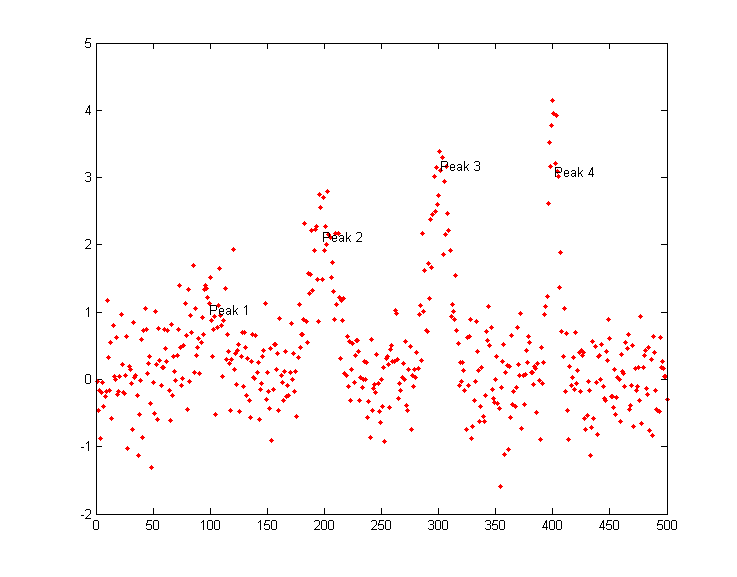a direct comparison of the accuracy of findpeaksG vs peakfit, run the demonstration script peakfitVSfindpeaks.m. This script generates four very noisy peaks of different heights and widths, then measures them in two different ways: first with findpeaksG.m (figure on the left) and then with peakfit.m, and compares the results. The peaks detected by findpeaksG are labeled "Peak 1", "Peak 2", etc. If you run this script several times, it will generate the same peaks but with independent samples of the random noise each time. You'll find that both methods work well most of the time, with peakfit giving smaller errors in most cases (because it uses all the points in each peak, not just the top part), but occasionally findpeaksG will miss the first (lowest) peak and rarely it will detect an 5th peak that is not really there. On the other hand, peakfit.m is constrained to fit 4 and only 4 peaks each time.

The demonstration script FindpeaksComparison compares the accuracy of findpeaksG and findpeaksL to several peak detection functions when applied to signals with multiple peaks and variable types and amounts of baseline and random noise.

###findpeaksb.m is a variant of findpeaksG.m that more accurately measures peak parameters by using iterative least-square curve fitting based on my peakfit.m function This yields better peak parameter values than findpeaksG alone for three reasons:
(1) it can be set for different peak shapes with the input argument 'PeakShape';
(2) it fits the entire peak, not just the top part; and
(3) it has provision for background subtraction (when the input argument "autozero" is set to 1, 2, or 3 - linear, quadratic, or flat, respectively).
This function works best with isolated peaks that do not overlap. For version 3, the syntax is P = findpeaksb(x,y, SlopeThreshold, AmpThreshold, smoothwidth, peakgroup, smoothtype, windowspan, PeakShape, extra, AUTOZERO). The first seven input arguments are exactly the same as for the findpeaksG.m function; if you have been using findpeaksG or iPeak to find and measure peaks in your signals, you can use those same input argument values for findpeaksb.m. The remaining four input arguments of are for the peakfit function:
• "windowspan" specifies the number of data points over which each peak is fit to the model shape (This is the hardest one to estimate; in autozero modes 1 and 2, 'windowspan' must be large enough to cover the entire single peak and get down to the background on both sides of the peak, but not so large as to overlap neighboring peaks);
• "PeakShape" specifies the model peak shape: 1=Gaussian, 2=Lorentzian, etc (type 'help findpeaksb' for a list),
• "extra" is the shape modifier variable that is used for the Voigt, Pearson, exponentially broadened Gaussian and Lorentzian, Gaussian/Lorentzian blend, and bifurcated Gaussian and Lorentzian shapes to fine-tune the peak shape;
• "AUTOZERO" is 0, 1, 2, or 3 for no, linear, quadratic, or flat background subtraction.
The peak table returned by this function has a 6th column listing the percent fitting errors for each peak. Here is a simple example with three Gaussians on a linear background, comparing (a) plain findpeaksG, to (b)  findpeaksb without background subtraction (AUTOZERO=0), and to (c) findpeaksb with background subtraction (AUTOZERO=1).

x=1:.2:100;Heights=[1 2 3];Positions=[20 50 80];Widths=[3 3 3];
y=2-(x./50)+modelpeaks(x,3,1,Heights,Positions,Widths)+.02*randn(size(x));
plot(x,y);

disp('          Peak      Position      Height      Width        Area         % error')
PlainFindpeaks=findpeaksG(x,y,.00005,.5,30,20,3)
NoBackgroundSubtraction=findpeaksb(x,y,.00005,.5,30,20,3,150,1,0,0)
LinearBackgroundSubtraction=findpeaksb(x,y,.00005,.5,30,20,3,150,1,0,1)

The demonstration script DemoFindPeaksb.m shows how findpeaksb works with multiple peaks on a curved background, and FindpeaksComparison shows how findpeaksb compares to the other peak detection functions when applied to signals with multiple peaks and variable types and amounts of baseline and random noise.

findpeaksSb.m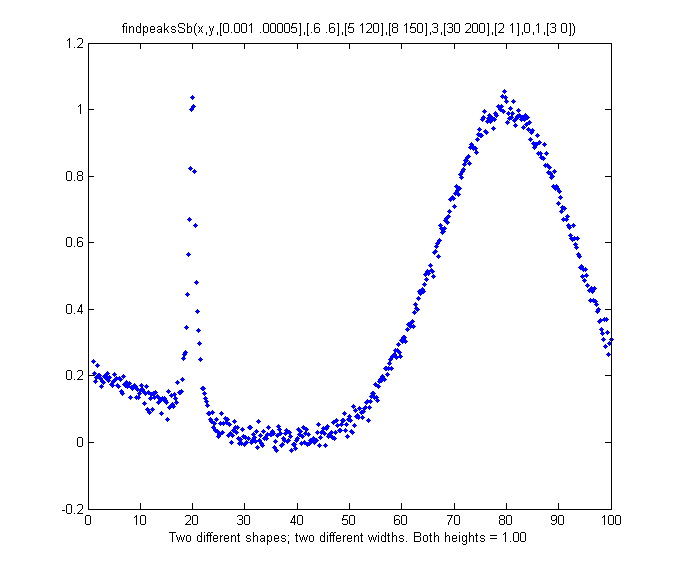is a segmented variant of findpeaksb.m. It has the same syntax as findpeaksb.m, except that the input arguments SlopeThreshold, AmpThreshold, smoothwidth, peakgroup, window, width, PeakShape, extra, NumTrials, autozero, and fixedparameters, can all optionally be scalars or vectors with one entry for each segment, in the same manner as findpeaksSG.m. Returns a matrix P listing the peak number, position, height, width, area, percent fitting error and "R2" of each detected peak. In the example on the right, the two peaks have the same height above baseline (1.00) but different shapes (the first Lorentzian and the second Gaussian), very different widths, and different baselines. So, using findpeaksG or findpeaksL or findpeaksb, it would be impossible to find one set of input arguments that would be optimum for both peaks.  But, using findpeaksSb.m, different settings can apply to different regions of the signal. In this simple example, there are only two segments, defined by SlopeThreshold with 2 different values, and the other input arguments are either the same or are different in those two segments. The result is that the peak height of the both peaks is measured accurately.

SlopeThreshold=
[.001 .00005]; AmpThreshold=.6; smoothwidth=[5 120]; peakgroup=[5 120];
smoothtype=3; window=[30 200]; PeakShape=[2 1]; extra=0; NumTrials=1; autozero=[3 0];

findpeaksSb(x,y, SlopeThreshold, AmpThreshold, smoothwidth, peakgroup, smoothtype, window, PeakShape, extra, NumTrials, autozero)

Peak #   Position   Height   Width    Area

1      19.979    0.9882    1.487    1.565
2      79.805    1.0052   23.888   25.563

DemoFindPeaksSb.m demonstrates the findpeaksSG.m function by creating a random number of Gaussian peaks whose widths increase by a factor of 25-fold over the x-axis range and that are superimposed on a curved baseline with random white noise that increases gradually; four segments are used in this example, changing the peak detection and curve fitting values so that all the peaks are measured accurately. GraphicPrintout.

###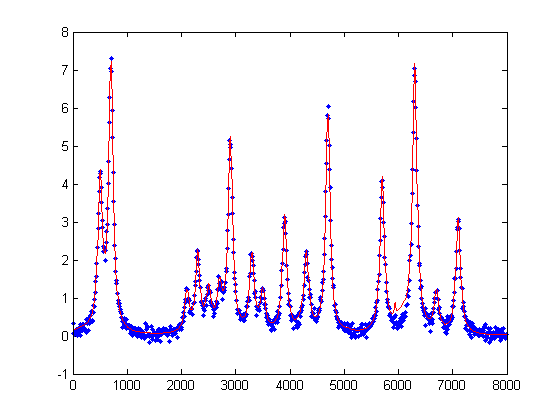findpeaksb3.m is a more ambitious variant of findpeaksb.m that fits each detected peak along with the previous and following peaks found by findpeaksG.m, so as to deal better with overlap of the adjacent overlapping peaks. The syntax is function FPB=findpeaksb3(x,y, SlopeThreshold, AmpThreshold, smoothwidth, peakgroup, smoothtype, PeakShape, extra, NumTrials, AUTOZERO, ShowPlots). The demonstration script DemoFindPeaksb3.m shows how findpeaksb3 works with irregular clusters of overlapping Lorentzian peaks, as in the example on the left (type "help findpeaksb3") for more. The demonstration script FindpeaksComparison shows how findpeaksb3 compares to the other peak detection functions when applied to signals with multiple peaks and variable types and amounts of baseline and random noise.

###findpeaksfit.m is essentially a serial combination of findpeaksG.m and peakfit.m. It uses the number of peaks found and the peak positions and widths determined by findpeaksG as input for the peakfit.m function, which then fits the entire signal with the specified peak model. This combination yields better values than findpeaksG alone, because peakfit fits the entire peak, not just the top part, and it deals with non-Gaussian and overlapped peaks. However, it fits only those peaks that are found by findpeaksG. The syntax is

function [P,FitResults,LowestError,BestStart,xi,yi] =
findpeaksfit(x, y, SlopeThreshold, AmpThreshold, smoothwidth, peakgroup, smoothtype, peakshape, extra, NumTrials, autozero, fixedparameters, plot
s)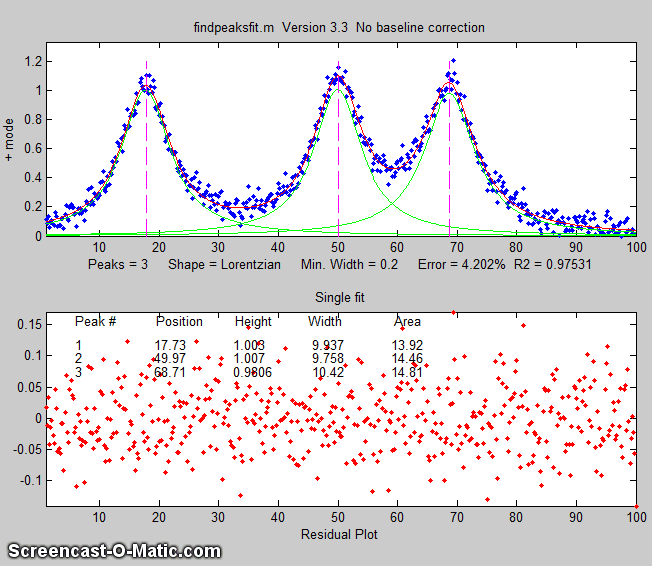The first seven input arguments are exactly the same as for the findpeaksG.m function; if you have been using findpeaksG or iPeak to find and measure peaks in your signals, you can use those same input argument values for findpeaksfit.m. The remaining six input arguments of findpeaksfit.m are for the peakfit function; if you have been using peakfit.m or ipf.m to fit the peaks in your signals, then you can use those same input argument values for findpeaksfit.m. The demonstration script findpeaksfitdemo.m, shows findpeaksfit automatically finding and fitting the peaks in a set of 150 signals, each of which may have 1 to 3 noisy Lorentzian peaks in variable locations, artificially slowed down with the "pause" function so you can see it better. Requires the findpeaksfit.m and lorentzian.m functions installed. This script was used to generate the GIF animation shown on the left. Type "help findpeaksfit" for more information.

Which to use: findpeaksG/L, findpeaksb, findpeaksb3, or findpeaksfit? The demonstration script FindpeaksComparison.m compares the peak parameter accuracy all four of those peak detection functions applied to a computer-generated signal with multiple peaks plus variable types and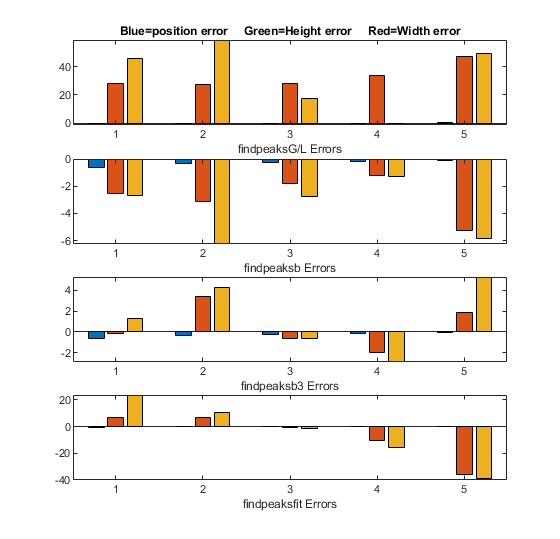amounts of baseline and random noise. (Requires those four functions, plus gaussian.m, lorentzian.m, modelpeaks.m, findpeaksG.m, findpeaksL.m, pinknoise.m, and propnoise.m, in the Matlab/Octave path). Results are displayed graphically in figure windows 1, 2, and 3 and printed out in a table of parameter accuracy and elapsed time for each method, as shown below. You may change the lines in the script marked by <<< to modify the number and character and amplitude of  the signal peaks, baseline, and noise. (Make the signal similar to yours to discover which method works best for your type of signal). The best method depends mainly on the shape and amplitude of the baseline and on the extent of peak overlap. Type "help FindpeaksComparison" for details.

###Average absolute percent errors of all peaks
Position error  Height error  Width error  Elapsed time, sec
findpeaksG     0.35955     38.5733       25.7977      0.17447
findpeaksb     0.388283     8.50246      14.3295      0.78245
findpeaksb3    0.27187      3.7445        3.0474      1.2146
findpeaksfit   0.519302     8.04176      24.035       1.017

Note:
findpeaksfit.m differs from findpeaksb.m in that findpeaksfit.m fits all the found peaks at one time with a single multi-peak model, whereas findpeaksb.m fits each peak separately with a single-peak model, and findpeaksb3.m fits each detected peak along with the previous and following peaks. As a result, findpeaksfit.m works better with a relatively small number of peak that all overlap, whereas findpeaksb.m works better with a large number of isolated non-overlapping peaks, and findpeaksb3.m works for large numbers of peaks that overlap at most one or two adjacent peaks. FindpeaksG/L is simple and fast, but it does not perform baseline correction; findpeaksfit can perform flat, linear, or quadratic baseline correction, but it works only over the entire signal at once; in contrast, findpeaksb and findpeaksb3 perform local baseline correction, which often works well if the baseline is curved or irregular.

Other related functions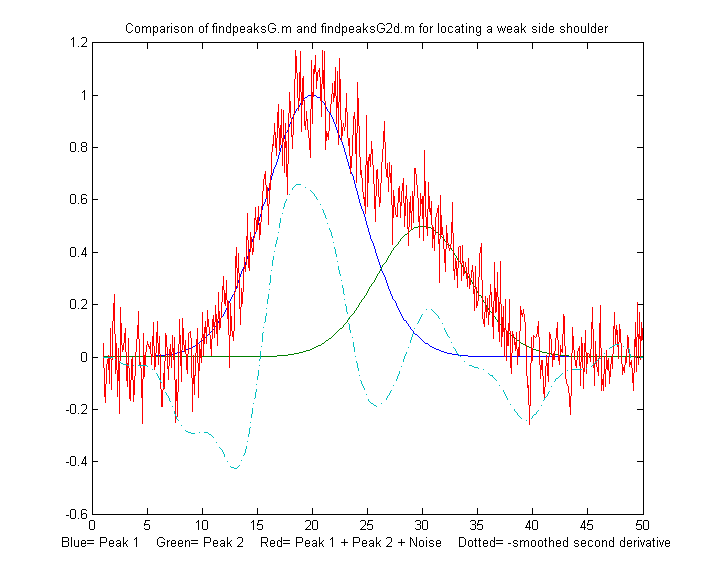findpeaksG2d.m is a variant of findpeaksSG that can be used to locate the positive peaks and shoulders in a noisy x-y time series data set. Detects peaks in the negative of the second derivative of the signal, by looking for downward slopes in the third derivative that exceed SlopeThreshold. See TestFindpeaksG2d.m.

[M,A]=autopeaks.m is a peak detector for peaks of arbitrary shape; it's basically a combination or autofindpeaks.m and measurepeaks.m. It has similar syntax to measurepeaks.m, except that the peak detection parameters (SlopeThreshold, AmpThreshold, smoothwidth  peakgroup, and smoothtype) can be omitted and the function will calculate trial values in the manner of autofindpeaks.m. Using the simple syntax [M,A]=autopeaks(x, y) works well in some cases, but if not try [M,A]=autopeaks(x, y, n), using different values of n (roughly the number of  peaks that would fit into the signal record) until it detects the peaks that you want to measure. Like measurepeaks, it returns a table M containing the peak number, peak position, absolute peak height, peak-valley difference, perpendicular drop area, and tangent skim area of each peak it detects, but is also can optionally return a vector A containing the peak detection parameters that it calculates (for use by other peak detection and fitting functions). For the most precise control over peak detection, you can specify all the peak detection parameters by typing M=autopeaks(x,y, SlopeThreshold, AmpThreshold, smoothwidth, peakgroup). [M,A]=autopeaksplot.m is the same but it also plots the signal and the individual peaks in the manner of measurepeaks.m (shown above). The script testautopeaks.m runs all the examples in the autopeaks help file, with a 1-second pause between each one, printing out results in the command window and additionally plotting and numbering the peaks (Figure window 1) and each individual peak (Figure window 2); it requires gaussian.m and fastsmooth.m in the path.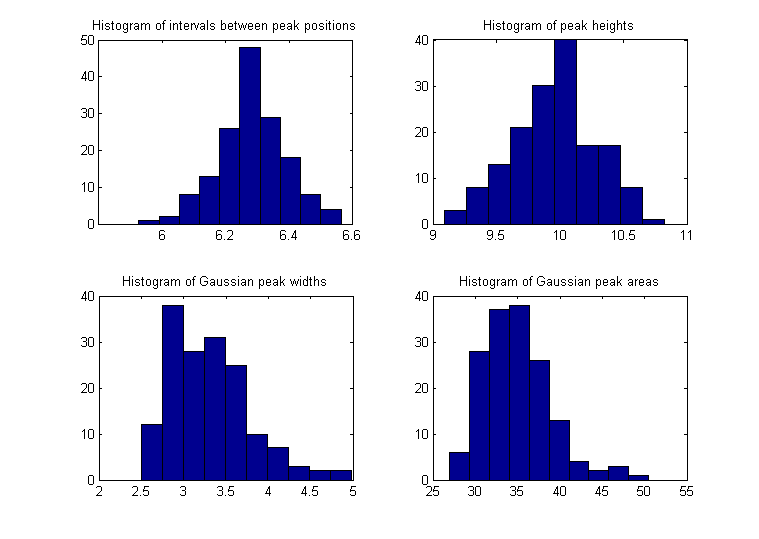The function peakstats.m uses the same algorithm as findpeaksG, but it computes and returns a table of summary statistics of the peak intervals (the x-axis interval between adjacent detected peaks), heights, widths, and areas, listing the maximum, minimum, average, and percent standard deviation of each, and optionally plotting the x,y data with numbered peaks in figure window 1, printing the table of peak statistics in the command window, and plotting the histograms of the peak intervals, heights, widths, and areas in the four quadrants of figure window 2. Type "help peakstats". The syntax is the same as findpeaksG, with the addition of a 8th input argument to control the display and plotting.
Version 2, March 2016, adds median and mode. Example:

x=[0:.1:1000];y=5+5.*cos(x)+randn(size(x));
PS=peakstats(x,y,0,-1,15,23,3,1);

Peak Summary Statistics
158 peaks detected

Interval     Height     Width        Area
Maximum    6.6428      10.9101     5.6258      56.8416
Minimum    6.0035      9.1217      2.5063      28.2559
Mean       6.283       9.9973      3.3453      35.4737
% STD      1.8259      3.4265      15.1007     12.6203
Median     6.2719      10.0262     3.2468      34.6473
Mode       6.0035      9.1217      2.5063      28.2559

With the last input argument omitted or equal to zero, the plotting and printing in the command window are omitted; the numerical values of the peak statistics table are returned as a 4x4 array, in the same order as the example above.

tablestats.m (PS=tablestats(P,displayit)) is similar to peakstats.m except that it accepts as input a peak table P such as generated by findpeaksG.m, findvalleys.m, findpeaksL.m, findpeaksb.m, findpeaksplot.m, findpeaksnr.m, findpeaksGSS.m, findpeaksLSS.m, or findpeaksfit.m - any of the functions that return a table of peaks with at least 4 columns listing peak number, height, width, and area. Computes the peak intervals (the x-axis interval between adjacent detected peaks) and the maximum, minimum, average, and percent standard deviation of each, and optionally displaying the histograms of the peak intervals, heights, widths, and areas in figure window 2. Set the optional last argument displayit = 1 if the histograms are to be displayed, otherwise not. Example:

x=[0:.1:1000];y=5+5.*cos(x)+.5.*randn(size(x));
figure(1);P=findpeaksplot(x,y,0,8,11,19,3);tablestats(P,1);

###FindpeaksE.m is a variant of findpeaksG.m that additionally estimates the percent relative fitting error of each peak (assuming a Gaussian peak shape) and returns it in the 6th column of the peak table.  Example:

>> x=[0:.01:5];
>> y=x.*sin(x.^2).^2+.1*whitenoise(x);
>> P=findpeaksE(x,y,.0001,1,15,10)
P =
1    1.3175     1.3279     0.25511    0.36065    5.8404
2    1.4245     1.2064     0.49053    0.62998   10.476
3    2.1763     2.1516     0.65173    1.4929     3.7984
4    2.8129     2.8811     0.2291     0.70272    2.3318...

###Peak start and end.  Defining the "start" and "end" of the peak (the x-values where the peak begins and ends) is a bit arbitrary because typical peak shapes approach the baseline asymptotically far from the peak maximum. You might define the peak start and end points as the x values where the y value is some small fraction, say 1%, of the peak height, but then the random noise on the baseline is likely to be a large fraction of the signal amplitude at that point. Smoothing to reduce noise is likely to distort and broaden peaks, effectively changing their start and end points. Overlap of peaks also greatly complicates the issue. One solution is to fit each peak to a model shape, then calculate the peak start and end from the model expression. That method minimizes the noise problem by fitting the data over the entire peak, and it can handle overlapping peaks, but it works only if the peaks can be modeled by available fitting programs. For example, Gaussian peaks can be shown to reach a fraction a of the peak height at x = p±sqrt(w^2 log(1/a))/(2 sqrt(log(2))) where p is the peak position and w is the peak width (full width at half maximum).  So, for example if a = .01, x = p±w*sqrt((log(2)+log(5))/(2 log(2))) = 1.288784*w. Lorentzian peaks can be shown to reach a fraction a of the peak height at x = p±sqrt[(w^2 - a w^2)/a]/2. If a = .01, x = p±(3/2 sqrt(11)*w) = 4.97493*w. The findpeaksG variants findpeaksGSS.m and findpeaksLSS.m, for Gaussian and Lorentzian peaks respectively, compute the peak start and end positions in this manner and return them in the 6th and 7th columns of the peak table P.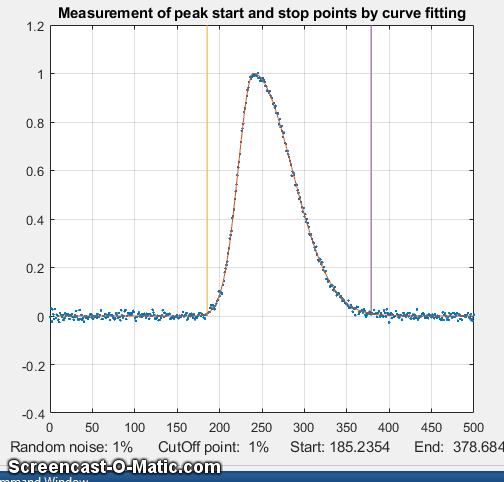The problem with this method is that it requires an analytical peak model, expressed as a closed-form expression, that can be solved algebraically for their start and end points. A more versatile method is to fit a model to the peak data by iterative curve fitting, and then use the best-fit model to locate the start and stop points by interpolation. For complex peak shapes, the model need not be limited to a single peak; very often complex peak shapes can be modeled as the sum of simple shapes, such as Gaussians. An example of this method is demonstrated in the script StartAndEnd.m, which simulates a noisy, asymmetrical peak and then applies this method using the downloadable peakfit.m function. You can select the start/stop cut-off point as a fraction of the peak height in line 8 and the amount or random noise in line 7. The default for both values is 0.01 (or 1%), which means that, at the cut-off points, the signal-to-noise ratio is very poor. Nevertheless, the start and end points can be calculated surprisingly precisely, because they are calculated from the best-fit model (contained in the output arguments xi and yi of the peakfit function), which averages out the noise over the entire signal (the more data points the better). The animation on the left shows the method in operation for 50 repeat measurements with different random noise samples, first with 1% noise and then with 10% noise. Despite the poor signal-to-noise ratio at the cut-off points, the relative standard deviation of the measured start and end times (marked by the vertical lines) is only about 0.2%. (Even when the noise is increased 10-fold to 0.1 in line 7, the results are still surprisingly good).

findpeaksT.m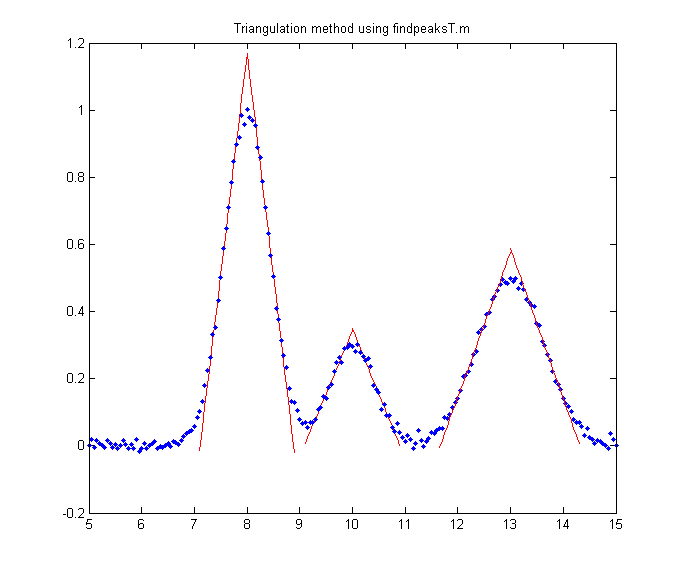and findpeaksTplot.m are variants of findpeaksG that measure the peak parameters by automatically constructing a triangle around each peak with its sides tangent to the sides of the peak, as shown on the right (script that generated this graphic). This method mimics the geometric construction method that was formerly used to measure peak parameters manually before the age of computers. Peak height is taken as the apex of the triangle, which is slightly higher than the peak of the underlying curve. The performance of this method is poor when the signals are very noisy or if the peaks overlap, but in a few circumstances the triangle construction method might be more accurate for the measurement of peak area than the Gaussian method, especially if the peaks are asymmetric or of uncertain shape (see the demo function triangulationdemo.m for some examples: click for graphic).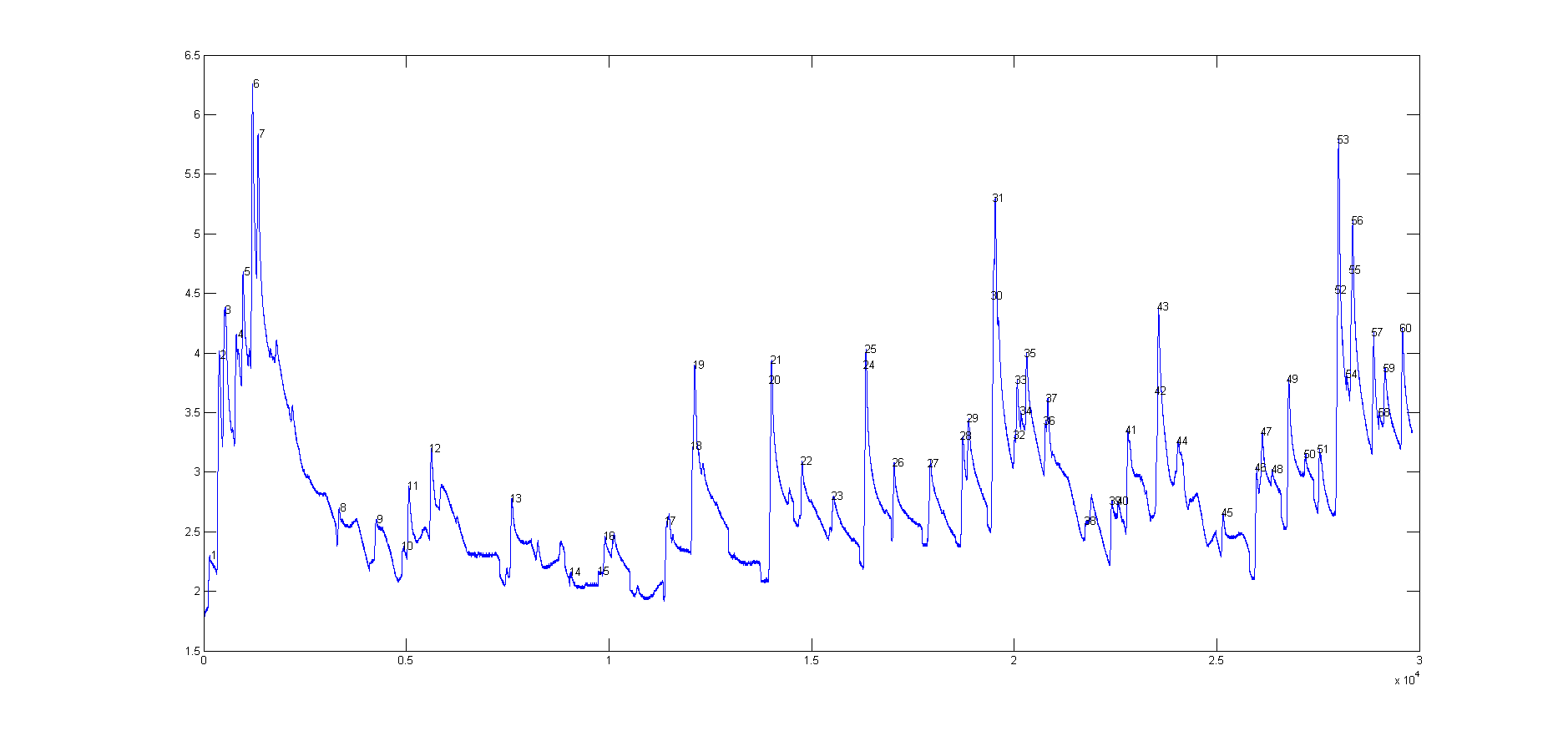findsteps.m, syntax: P=findpulses(x, y, SlopeThreshold, AmpThreshold, SmoothWidth, peakgroup), locates positive transient steps in noisy x-y time series data, by computing the first derivative of y that exceed SlopeThreshold, computes the step height as the difference between the maximum and minimum y values over a number of data point equal to "Peakgroup". Returns list (P) with step number, x position, y position, and the step height of each step detected; "SlopeThreshold" and "AmpThreshold" control step sensitivity; higher values will neglect smaller features.
Increasing "SmoothWidth" reduces small sharp false steps caused by random noise or by "glitches" in the data acquisition. See findsteps.png for a real example. And findstepsplot.m plots the signal and numbers the peaks.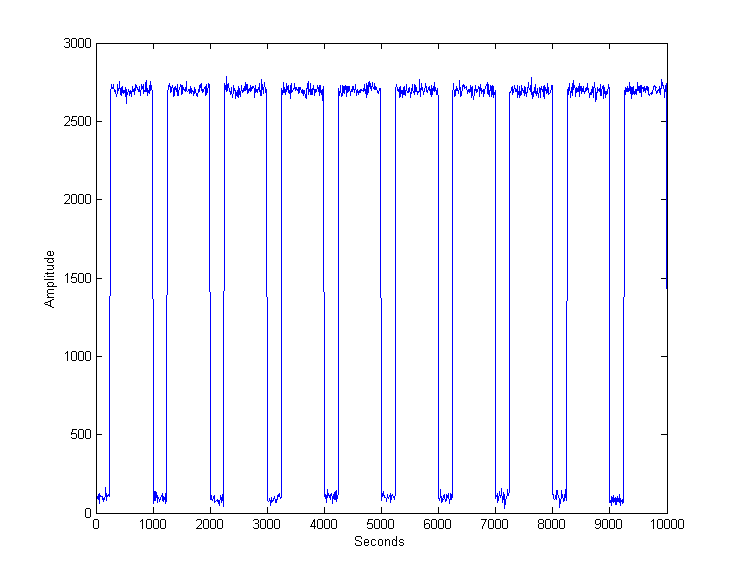Rectangular pulses (square waves) require a different approach, based on amplitude discrimination rather than differentiation. The function "findsquarepulse.m" (syntax S=findsquarepulse(t,y,threshold) locates the rectangular pulses in the signal t,y that exceed a y-value of "threshold" and determines their start time, average height (relative to the baseline) and width. DemoFindsquare.m creates a test signal (with a true height of 2636 and a height of 750) and calls findsquarepulse.m to demonstrate. If the signal is very noisy, some preliminary rectangular smoothing (e.g. using fastsmooth.m) before calling findsquarepulse.m may be helpful to eliminate false peaks.

NumAT(m,threshold): "Numbers Above Threshold": Counts the number of adjacent elements in the vector 'm' that are greater than or equal to the scalar value 'threshold'. Returns a matrix listing each group of adjacent values, their starting index, the number of elements in each group, the sum of each group, and the average (mean) of each group. Type "help NumAT" and try the example.

###Using the peak table
All these peak finding functions return a peak table as a matrix, with one row for each peak detected and with several columns listing, for example, the peak number, position, height, width, and area in columns 1 - 5 (with additional columns included for the variants measurepeaks.m, findpeaksnr.m,
findpeaksGSS.m, and findpeaksLSS.m). You can assign this matrix to a variable (e.g. P, in the examples above) and then use Matlab/Octave notation and built-in functions to extract specific information from that matrix. The powerful combination of functions and matrix/vector "colon" notation allows you to construct compact expressions that extract the very specific information that you need. For example:

[P(:,2) P(:,3)] is the time series of peak heights (peak position in the first column and peak height in the second column)
mean(P(:,3)) returns the average peak height of all peaks (because peak height is in column 3). Also works with median.
max(P(:,3)) returns the maximum peak height. Also works with min.
hist(P(:,3)) displays the histogram of peak heights.
std(P(:,4))./mean(P(:,4)) returns the relative standard deviation of the peak widths (column 4).

P(:,3)./max(P(:,3)) returns the ratio of each peak height (column 3) to the height of the highest peak detected.
100.*P(:,5)./sum(P(:,5)) returns the percentage of each peak area (column 5) of the total area of all peaks detected.

sortrows(P,2) sorts P by peak position; sortrows(P,3) sorts P by peak height (smallest to largest).

To create "d" as the vector of x-axis (position) differences between adjacent peaks (because peak position is in column 2).

for n=1:length(P)-1;d(n)=max(P(n+1,2)-P(n,2));end
(In Matlab/Octave, multiple statements can be placed on one line, separated by semicolons.)

The downloadable function [index,closestval] = val2ind(v,val) returns the index and the value of the element of vector 'v' that is closest to 'val' (download this function and place in the Matlab path). It's very useful in working with peak tables:
val2ind(P(:,3),7.5) returns the peak number of the peak whose height (column 3) is closest to 7.5
P(val2ind(P(:,2),7.5),3) returns the peak height (column 3) of the peak whose position (column 2) is closest to 7.5
P(val2ind(P(:,3),max(P(:,3))),:) returns the row vector of peak parameters of the highest peak in peak table P.
The three statements j=P(:,4)<5.8;k=val2ind(j,1);P(k,:)
return the matrix of peak parameters of all peaks in P whose widths (column 4) are less than 5.8.

### Demo scripts:  DemoFindPeak.m and DemoFindPeaksb.m

 DemoFindPeak.m is a simple demonstration script using the findpeaksG function on noisy synthetic data. Numbers the peaks and prints out the peak table in the Matlab command window:  ` Peak # Position Height Width AreaMeasuredpeaks = 1 799.95 6.9708 51.222 380.12 2 1199.4 3.9168 50.44 210.32 3 1600.6 2.9955 49.683 158.44 4 1800.4 2.0039 50.779 108.33 ......` DemoFindPeakSNR is a variant of DemoFindPeak.m that uses findpeaksnr.m to compute the signal-to-noise ratio (SNR) of each peak and returns it in the 5th column (click for graphic).DemoFindPeaksb.m is a similar demonstration script that uses the findpeaksb function on noisy synthetic data consisting of variable numbers of Gaussian peaks superimposed on a variable curved background.  (The findpeaksG function would not give accurate measurements of peak height, width, and area for this signal, because it does not correct for the background).   Relative Percent Errors    Position    Height    Width      Area   -0.0022464   0.54487   1.4057     1.9429   -0.02727     5.0091    8.9204    13.483    0.008429    -1.1224  -1.4923    -2.6315 .... % Root mean square errors ans =   0.044428   2.2571     3.8253     5.8501

## Peak Identification

The command line function idpeaks.m is used for identifying peaks according to their x-axis maximum positions, which is very useful in spectroscopy and in chromatography. The syntax is

[IdentifiedPeaks,AllPeaks]=idpeaks(DataMatrix, AmpT, SlopeT, SmoothWidth, FitWidth, maxerror, Positions, Names)

It finds peaks in the signal "DataMatrix" (x-values in column 1 and y-values in column 2), according to the peak detection parameters "AmpT", "SlopeT", "SmoothWidth", "FitWidth" (see the "findpeaksG" function above), then compares the found peak positions (x-values) to a database of known peaks, in the form of an array of known peak maximum positions ('Positions') and matching cell array of names ('Names'). If the position of a peak found in the signal is closer to one of the known peaks by less than the specified maximum error ('maxerror'), that peak is considered a match and its peak position, name, error, and peak amplitude (height) are entered into the output cell array "IdentifiedPeaks". The full list of detected peaks, identified or not, is returned in "AllPeaks". Use "cell2mat" to access numeric elements of IdentifiedPeaks,e.g. cell2mat(IdentifiedPeaks(2,1)) returns the position of the first identified peak, cell2mat(IdentifiedPeaks(2,2)) returns its name, etc. Obviously for your own applications, it's up to you to provide your own array of known peak maximum positions ('Positions') and matching cell array of names ('Names') for your particular types of signals. The related function idpeaktable.m does the same thing for a peak table P returned by any of my peak finder or peak fitting functions, having one row for each peak and columns for peak number, position, and height as the first three columns. The syntax is [IdentifiedPeaks] = idpeaktable(P,maxerror,Positions,Names). The interactive iPeak function described in the next section has this function built in as one of the keystroke commands.

Example:  Download idpeaks.zip, extract it, and place the extracted files in the Matlab or Octave path. This contains a high-resolution atomic emission spectrum of copper ('spectrum', x = wavelength in nanometers; y = amplitude) and a data table of known Cu I and Cu II atomic lines ('DataTable') containing the positions and names of many copper lines. The idpeaks function detects and measures the peak locations of all the peaks in "spectrum", then looks in
'DataTable' to see if any of those peaks are within .01 nm of any entry in the table and prints out the peaks that match.
`>> load DataTable>> load spectrum>> idpeaks(Cu,0.01,.001,5,5,.01,Positions,Names)ans=  'Position'   'Name'           'Error'         'Amplitude'  [  221.02]   'Cu II 221.027'  [ -0.0025773]   [ 0.019536]  [  221.46]   'Cu I 221.458'   [ -0.0014301]   [   0.4615]  [  221.56]   'Cu I 221.565'   [-0.00093125]   [  0.13191].................... etc...`

###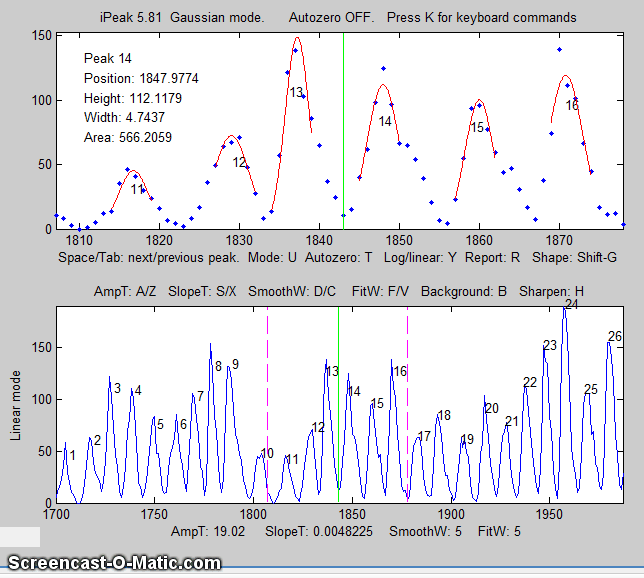Zoom control (up/down cursor arrows)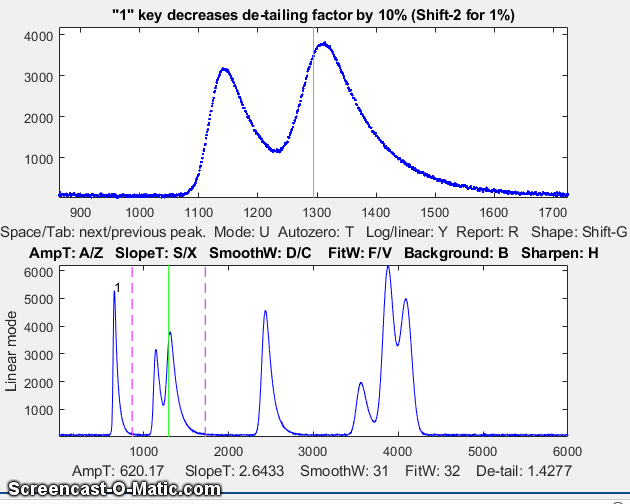Peak de-tailing (Shift-Y) version 8 or later.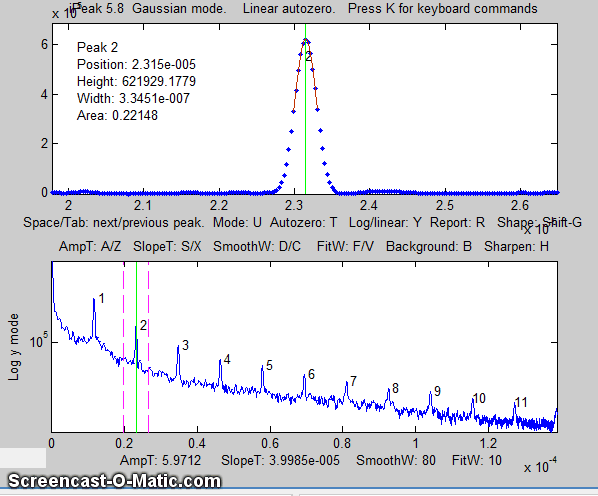Jump to Next/Previous peak (Space/Tab keys)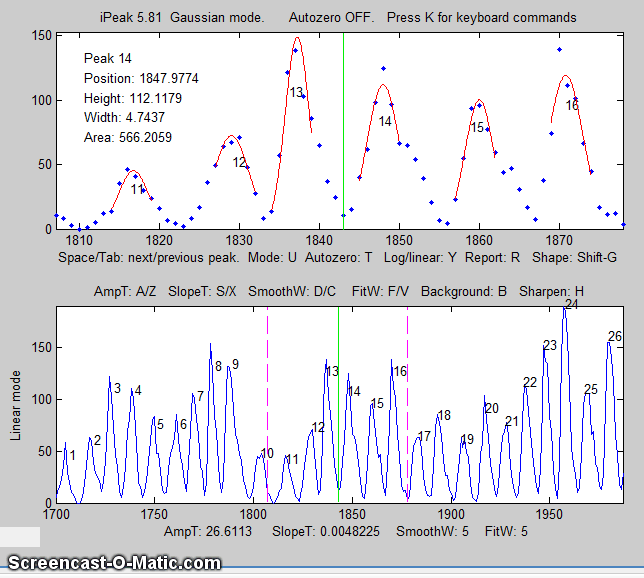Amplitude Threshold adjust (A/Z keys)
Click to enlarge
iPeak is a keyboard-operated interactive peak finder for time series data, based on the "findpeaksG.m" and "findpeaksL.m" functions, for Matlab only. Its basic operation is similar to iSignal and ipf.m. It accepts data in a single vector, a pair of vectors, or a matrix with the independent variable in the first column and the dependent variable in the second column. If you call iPeak with only those one or two input arguments, it estimates a default initial value for the peak detection parameters (AmpThreshold, SlopeThreshold, SmoothWidth, and FitWidth) based on the formulas below and displays those values at the bottom of the screen.
WidthPoints=length(y)/
20
SlopeThreshold=WidthPoints^-2;
AmpThreshold=abs(min(y)+0.1*(max(y)-min(y)));
SmoothWidth=round(WidthPoints/3);
FitWidth=round(WidthPoints/3);

You can then fine-tune the peak detection by using the A/Z, S/X, D/C, and F/V keys.
Added mode(y) subtraction as the 4th baseline correction mode selected by the T key. Previous version added measUrepeaks function (Shift-U) to display perpendicular drop and tangent skim area measurements. Added Shift-Y to enter de-tailing (sYmmetrization) factor for exponentially modified peaks, and 1 & 2 (and Shift-1 & Shift-2) to adjust.

Example 1:

One input argument; data in single vector:

>> y=cos(.1:.1:100);ipeak(y)

Example 2:  One input argument; data in two columns of a matrix:

>> x=[0:.01:5]';y=x.*sin(x.^2).^2;M=[x y];ipeak(M)
Example  3:  Two input arguments; data in separate x and y vectors:
>> x=[0:.1:100];y=(x.*sin(x)).^2;ipeak(x,y);

###Example 4When you start iPeak using the simple syntax above, the initial values of the peak detection parameters are calculated as described above, but if it starts off by picking up far too many or too few peaks, you can add an additional input argument (after the data) to control peak sensitivity.

>> x=[0:.1:100];y=5+5.*cos(x)+randn(size(x));ipeak(x,y,10);
or  >> ipeak([x;y],10);
or  >> ipeak(humps(0:.01:2),3)
or  >> x=[0:.1:10];y=exp(-(x-5).^2);ipeak([x' y'],1)

is an estimate of maximum peak density (PeakD), the ratio of the typical peak width to the length of the entire data record. Small values detect fewer peaks; larger values detect more peaks. It effects only the starting values for the peak detection parameters. (It's just a quick way to set reasonable initial values of the peak detection parameters, so you won't have so much adjusting to do).

>> ipeak(year,number,20)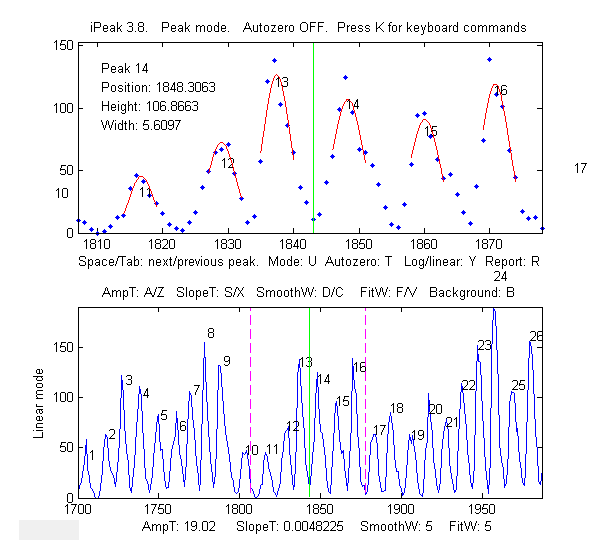iPeak displays the entire signal in the lower half of the Figure window and an adjustable zoomed-in section in the upper window. Pan and zoom the portion in the upper window using the cursor arrow keys.  The peak closest to the center of the upper window is labeled in the upper left of the top window, and it peak position, height, and width are listed. The Spacebar/Tab keys jump to the next/previous detected peak and displays it in the upper window at the current zoom setting (use the up and down cursor arrow keys to adjust the zoom range). Or you can press the J key to jump to a specified peak number.  Adjust the peak detection parameters AmpThreshold (A/Z keys), SlopeThreshold (S/X), SmoothWidth (D/C), FitWidth (F/V) so that it detects the desired peaks and ignores those that are too small, too broad, or too narrow to be of interest. You can also type in a specific value of AmpThreshold by pressing Shift-A or a specific value of SlopeThreshold by pressing Shift-S. Detected peaks are numbered from left to right.

Press P to display the peak table of all the detected peaks and their measurements by peak-top curve fitting (Peak #, Position, Height, Width, Area, and percent fitting error):

Gaussian shape mode (press Shift-G to change)
Window span: 169 units
Linear baseline subtraction
Peak#    Position    Height     Width      Area      Error
1      500.93      6.0585     34.446     222.17     9.5731
2      767.75      1.8841    105.58      211.77    25.979
3      1012.8      0.20158    35.914       7.7     269.21
4...........

Press Shift-U to measure the peaks by the perpendicular drop and tangent skim methods:

Peak Area Measurement (Shift-U key)
Peak   Position   PeakMax Peak-valley  Perp drop  Tan skim
1    300.19     12.011     9.3502     839.32     537.85
2    399.84     10.011     6.8604     700.83     363.15

3    498.67     1.9994    0.85312     125.29      31.4

4 ............

Press Shift-G to
cycle between Gaussian, Lorentzian, and flat-top shape modes. Press Shift-P to save peak table as disc file. Press U to switch between peak and valley mode. Don't forget that only valleys above (that is, more positive or less negative than) the AmpThreshold are detected; if you wish to detect valleys that have negative minima, then AmpThreshold must be set more negative than that.  Note: to speed up the operation for signals over 100,000 points in length, the lower window is refreshed only when the number of detected peaks changes or if the Enter key is pressed. Press K to see all the keystroke commands.
Press U key to switch between peak and valley mode.

If the density of data points on the peaks is too low - less than about 4 points - the peaks may not be reliably detected; you can improve reliability by using the interpolation command (Shift-I) to re-sample the data by linear interpolation to a larger number of points.  Conversely, if
the density of data points on the peaks of interest is very high - say, more than 100 points per peak - then you can speed up the operation of iPeak by re-sampling to a smaller number of points.

###Peak Summary Statistics. The E key prints a table of summary statistics of the peak intervals (the x-axis interval between adjacent detected peaks), heights, widths, and areas, listing the maximum, minimum, average, and percent standard deviation, and displaying the histograms of the peak intervals, heights, widths, and areas in figure window 2.

Peak Summary Statistics
149 peaks detected
No baseline correction
Interval      Height      Width          Area
Maximum    1.3204       232.7724    0.33408      80.7861
Minimum    1.1225       208.0581    0.27146      61.6991
Mean       1.2111       223.3685    0.31313      74.4764
% STD      2.8931       1.9115      3.0915       4.0858

Example 5: Six input arguments. As above, but input arguments 3 to 6 directly specifies initial values of AmpThreshold (AmpT), SlopeThreshold (SlopeT), SmoothWidth (SmoothW), FitWidth (FitW). PeakD is ignored in this case, so just type a '0' as the second argument after the data matrix).
>> ipeak(datamatrix,0,.5,.0001,20,20);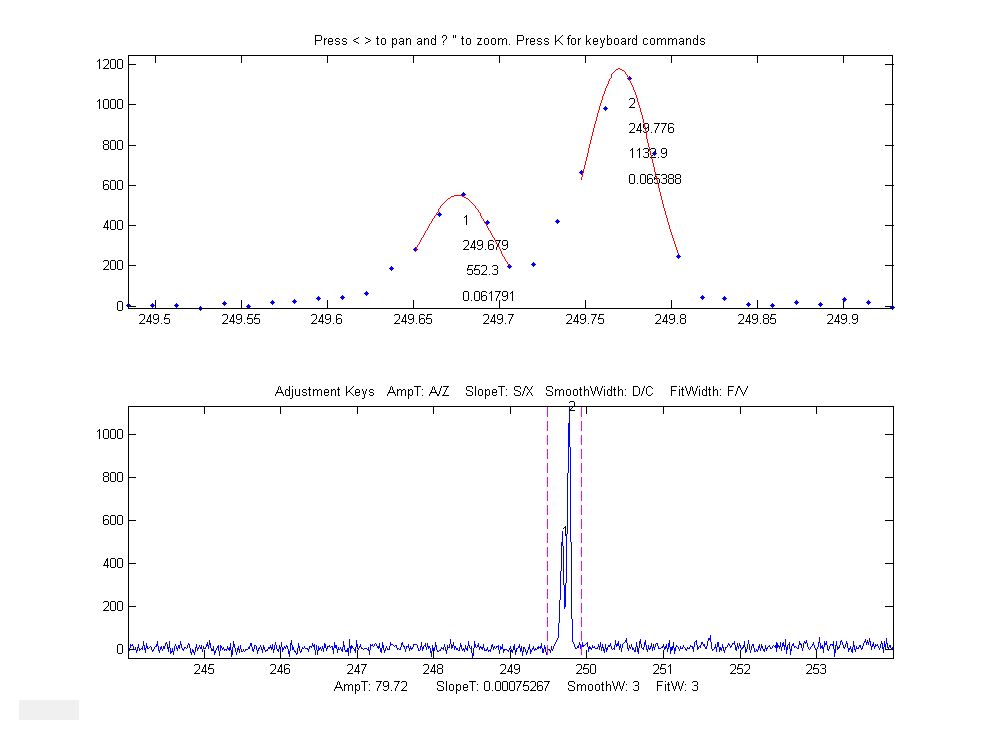Pressing 'L' toggles ON and OFF the peak labels in the upper window.

Keystrokes allow you to pan and zoom the upper window, to inspect each peak in detail if desired.  You can set the initial values of pan and zoom in optional input arguments 7 ('xcenter') and 8 ('xrange').  See example 6 below.

The Y key toggles between linear and log y-axis scale in the lower window (a log axis is good for inspecting signals with high dynamic range). It effects only the lower window display and has no effect on the data itself or on the peak detection and measurements.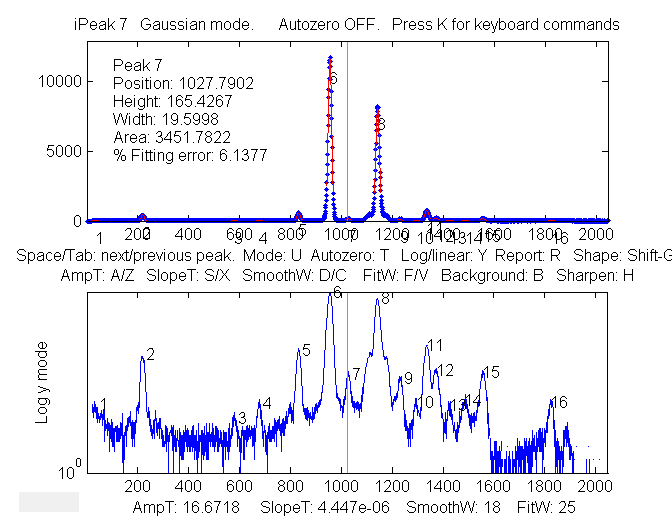The Y key displays the signal on a Log scale in the lower window, which makes the smaller peaks easier to see.

Example 6: Eight input arguments. As above, but input arguments 7 and 8 specify the initial pan and zoom settings, 'xcenter' and 'xrange', respectively.  In this example, the x-axis data are wavelengths in nanometers (nm), and the upper window zooms in on a very small 0.4 nm region centered on 249.7 nm. (These data, provided in the ZIP file, are from a high-resolution atomic spectrum).

>> ipeak(Sample1,0,100,0.05,3,4,249.7,0.4);

Baseline correction
methods. The T key cycles the baseline correction mode from off, linear, quadratic, flat, linear mode(y), flat mode(y), and then back to off. The current mode is displayed above the upper panel. When the baseline correction is OFF, peak heights are measured relative to zero. (Use this method mode when the baseline is zero or if you have previously subtracted the baseline from the entire signal using the B key). In the linear or quadratic methods, peak heights are automatically measured relative to the local baseline interpolated from the points at the ends of the segment displayed in the upper panel; use the zoom controls to isolate a group of peaks so that the signal returns to the local baseline at the beginning and end of the segment displayed in the upper window. The peak heights, widths, and areas in the peak table (R or P keys) will be automatically corrected for the baseline. The linear or quadratic methods will work best if the peaks are well separated so that the signal returns to the local baseline between the peaks.  (If the peaks are highly overlapped, or if they are not Gaussian in shape, the best results will be obtained by using the curve fitting function - the N or M keys. The 3rd method flat is used only for curve fitting function, to account for a flat baseline offset without reference to the edges of the signal segment being fit). The mode(y) methods 4 and 5 subtract the most common y value (the statistical "mode") from all the points in the selected region. For peak-type signals where the peaks often return to the baseline between peaks, the most common value is usually a good approximation of the baseline even if the signal does not return to the baseline at the ends as required by methods 2 and 3 (graphic example).

Example 7:  Nine input arguments. As example 6, but the 9th input argument sets the background correction mode (equivalent to pressing the T key)' 0=OFF; 1=linear; 2=quadratic, 3=flat, 4=mode(y).  If not specified, it is initially OFF.

>> ipeak(Sample1,0,100,0.00,3,4,249.7,0.4,1);

Converting to command-line functions.  To aid in writing your own scripts and function to automate processing, the 'Q' key prints out the findpeaksG, findpeaksb, and findpeaksfit commands for the segment of the signal in the upper window and for the entire signal, with most or all of the input arguments in place, so you can Copy and Paste into your own scripts. The 'W' key similarly prints out the peakfit and ipf commands. This provides a way to deal with signals that require different signal processing in different regions of their x-axis ranges, by allowing you to create a series of command-line functions for each local region that, when executed in sequence, quickly process each segment of the signal appropriately.

Shift-Ctrl-S transfers the current signal to iSignal.m and Shift-Ctrl-P  transfers the current signal to Interactive Peak Detector (iPeak.m), if those functions are installed in your Matlab path.

###Ensemble averaging. For signals that contain repetitive waveform patterns occurring in one continuous signal, with nominally the same shape except for noise, the ensemble averaging function (Shift-E) can compute the average of all the repeating waveforms. It works by detecting a single peak in each repeat waveform in order to synchronize the repeats (and therefore does not require that the repeats be equally spaced or synchronized to an external reference signal). To use this function, first adjust the peak detection controls to detect only one peak in each repeat pattern, then zoom in to isolate any one of those repeat patterns, and then press Shift-E. The average waveform is displayed in Figure 2 and saved as “EnsembleAverage.mat” in the current directory. See iPeakEnsembleAverageDemo.m.De-tailing peaks with exponential tails.  If your signal has peaks tail that to the right or left because they have been exponentially broadened,  you can remove the tails by the first-derivative addition technique described previously. To activate this process, press Shift-Y, enter a rough estimate of the exponential time constant, and then use the 1 and 2 keys to adjust the de-tailing factor by 10% (or Shift-1 and Shift-2 to adjust by 1%). Increase the factor until the baseline after the peak goes negative, then increase it slightly so that it is as low as possible but not negative.  (It the peaks tail to the left rather than the right, use a negative factor). This results in narrower, taller peaks, and it works with any peak shape that has been exponentially broadened. It has the same effect as deconvoluting the exponential function from the broadened peak, but it is faster and simpler. To cancel the effect, press Shift-Y and set the time constant to zero.

Normal and Multiple Peak fitting: The N key applies iterative curve fitting to the detected peaks that are displayed in the upper window (referred to here as "Normal" curve fitting). The use of the iterative least-squares function can result in more accurate peak parameter measurements than the normal peak table (R or P keys), especially if the peaks are non-Gaussian in shape or are highly overlapped. (If the peaks are superimposed on a background, select the baseline correction mode using the T key, then use the pan and zoom keys to select a peak or a group of overlapping peaks in the upper window, with the signal returning all the way to the local baseline at the ends of the upper window if you are using the linear or quadratic baseline modes).  Make sure that the AmpThreshold, SlopeThreshold, SmoothWidth are adjusted so that each peak is numbered once. Only numbered peaks are fit. Then press the N key, type a number for the desired peak shape from the menu displayed in the Command window (iPeak 7.9):

Gaussians: y=exp(-((x-pos)./(0.6005615.*width)) .^2)
Gaussians with independent positions and widths...................1 (default)
Gaussians with the same widths....................................6
Gaussians with preset fixed widths...............................11
Fixed-position Gaussians.........................................16
Asymmetrical Gaussians with unequal half-widths on both sides....14
Lorentzians: y=ones(size(x))./(1+((x-pos)./(0.5.*width)).^2)
Lorentzians with independent positions and widths.................2
Equal-width Lorentzians...........................................7
Fixed-width Lorentzian...........................................12
Fixed-position Lorentzian........................................17
Gaussian/Lorentzian blend (equal blends)...........................13
Fixed-width Gaussian/Lorentzian blend............................35
Gaussian/Lorentzian blend with independent blends)...............33
Voigt profile with equal alphas)...................................20
Fixed-width Voigt profile with equal alphas......................34
Voigt profile with independent alphas............................30
Logistic: n=exp(-((x-pos)/(.477.*wid)).^2); y=(2.*n)./(1+n).........3
Pearson: y=ones(size(x))./(1+((x-pos)./((0.5.^(2/m)).*wid)).^2).^m..4
Fixed-width Pearson..............................................37
Pearson with independent shape factors, m........................32
Breit-Wigner-Fano..................................................15
Exponential pulse: y=(x-tau2)./tau1.*exp(1-(x-tau2)./tau1)..........9
Alpha function: y=(x-spoint)./pos.*exp(1-(x-spoint)./pos);.........19
Up Sigmoid (logistic function): y=.5+.5*erf((x-tau1)/sqrt(2*tau2)).10
Down Sigmoid y=.5-.5*erf((x-tau1)/sqrt(2*tau2))....................23
Triangular.........................................................21

and press Enter
, then type in a number of repeat trial fits and press Enter (the default is 1; start with that and then increase if necessary).  If you have selected a variable-shape peak (e.g. numbers 4, 5, 8 ,13, 14, 15, 18, 20, 30-33), the program will ask you to type in a number that fine-tunes the shape. The program will then perform the fit, display the results graphically in Figure window 2, and print out a table of results in the command window, e.g.:

Peak shape (1-8): 2
Number of trials: 1

Least-squares fit to Lorentzian peak model
Fitting Error 1.1581e-006%
Peak#   Position   Height   Width   Area
1        100        1       50    71.652
2        350        1      100    146.13
3        700        1      200    267.77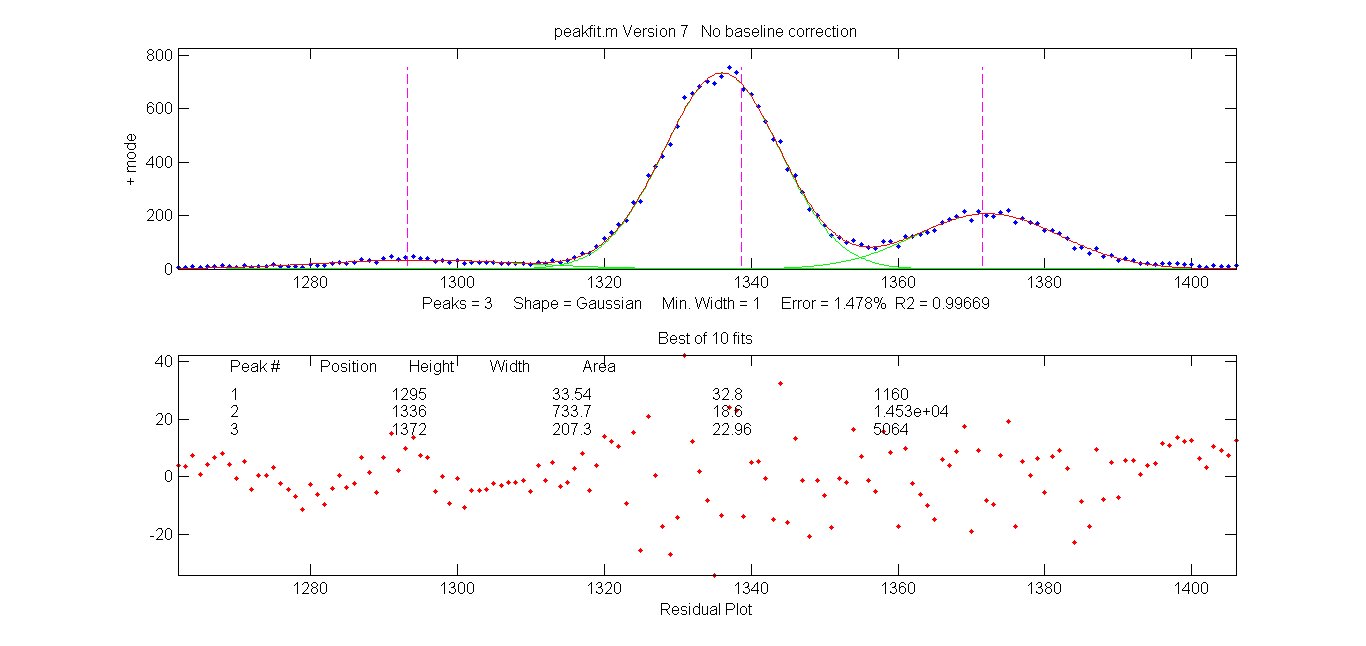Normal Peak Fit (N key) applied to a group of three overlapping Gaussians peaks

###There is also a "Multiple" peak fit function (M key) that will attempt to apply iterative curve fitting to all the detected peaks in the signal simultaneously. Before using this function, it's best to turn off the automatic baseline correction (T key) and use the multi-segment baseline correction function (B key) to remove the background (because the baseline correction function will probably not be able to subtract the baseline from the entire signal). Then press M and proceed as for the normal curve fit. A multiple curve fit may take a minute or so to complete if the number of peaks is large, possibly longer than the Normal curve fitting function on each group of peaks separately.

The N and M key fitting functions perform non-linear iterative curve fitting using the peakfit.m function. The number of peaks and the starting values of peak positions and widths for the curve fit function are automatically supplied by the the findpeaksG function, so it is essential that the peak detection variables in iPeak be adjust so that all the peaks in the selected region are detected and numbered once.  (For more flexible curve fitting, use ipf.m, which allows manual optimization of peak groupings and start positions).

Example 8.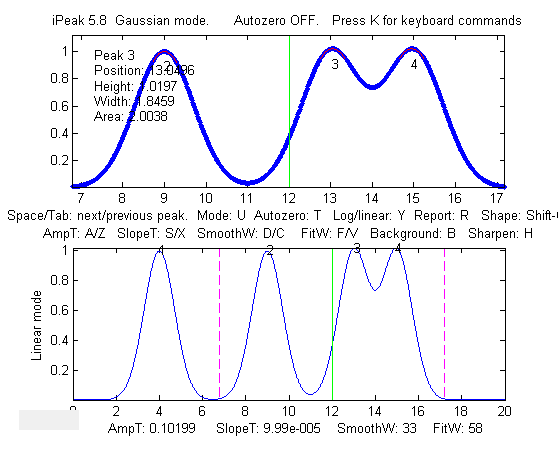This example generates four Gaussian peaks, all with the exact same peak height (1.00) and area (1.773). The first peak (at x=4) is isolated, the second peak (x=9) is slightly overlapped with the third one, and the last two peaks (at x= 13 and 15) are strongly overlapped.

x=[0:.01:20];
y=exp(-(x-4).^2)+exp(-(x-9).^2)+exp(-(x-13).^2)+exp(-(x-15).^2);
ipeak(x,y)

By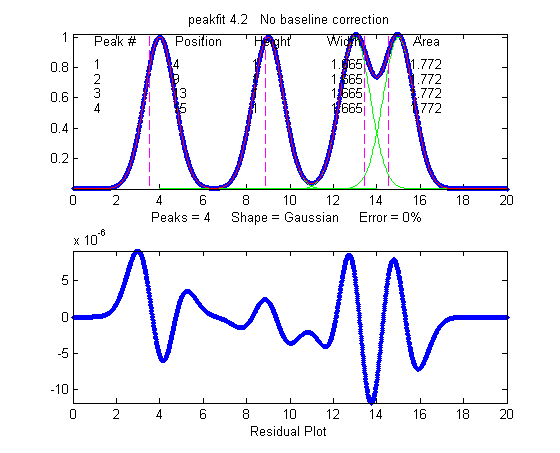itself, iPeak does a fairly good just of measuring peaks positions and heights by fitting just the top part of the peaks, because the peaks are Gaussian, but the areas and widths of the last two peaks (which should be 1.665 like the others) are quite a bit too large because of the overlap:
Peak#   Position    Height    Width     Area
1        4          1       1.6651     1.7727
2        9          1       1.6651     1.7727

3       13.049      1.0
2    1.8381     1.9956
4       14.951      1.0
2    1.8381     1.9956

In this case, curve fitting (using the N or M keys) does a much better job,
even if the overlap is even greater, but only if the peak shape is known:

Peak#    Position    Height    Width    Area
1         4          1       1.6651    1.7724
2         9          1       1.6651    1.7725
3        13          1       1.6651    1.7725
4        15         0.99999  1.6651    1.7724

• Note 1: If the peaks are too overlapped to be detected and numbered separately, try pressing the H key to activate the sharpen function before pressing M (version 4.0 and above only). This does not effect the signal itself, only the peak detection.

• Note 2If you plan to use a variable-shape peak (numbers 4, 5, 8 ,13, 14, 15, 18, or 20) for the Multiple peak fit, it's a good idea to obtain a reasonable value for the requested "extra" shape parameter by performing a Normal peak fit on an isolated single peak (or small group of partly-overlapping peaks) of the same shape, then use that value for the Multiple curve fit of the entire signal.

• Note 3: If the peak shape varies across the signal, you can either use the Normal peak fit to fit each section with a different shape rather than the Multiple peak fit, or you can use the unconstrained variable shapes that fit the shape individually for each peak: Voigt (30), ExpGaussian (31), Pearson (32), or Gaussian/Lorentzian blend (33).

Peak identification. There is an optional "peak identification" function if optional input arguments 9
('MaxError'), 10 ('Positions'), and 11 ('Names') are included. The "I" key toggles this function ON and OFF. This function compares the found peak positions (maximum x-values) to a reference database of known peaks, in the form of an array of known peak maximum positions ('Positions') and matching cell array of names ('Names'). If the position of a found peak in the signal is closer to one of the known peaks by less than the specified maximum error ('MaxError'), then that peak is considered a match and its name is displayed next to the peak in the upper window. When when the 'O' key is pressed (the letter 'O'), the peak positions, names, errors, and amplitudes are printed out in a table in the command window.

Example 9: Eleven input arguments. As above, but also specifies 'MaxError', 'Positions', and 'Names' in optional input arguments 9, 10, and 11, for peak identification function. Pressing the 'I' key toggles off and on the peak identification labels in the upper window.These data (provided in the ZIP file) are from a high-resolution atomic spectrum (x-axis in nanometers).

>> ipeak(Sample1,0,100,0.05,3,6,296,5,0.1,Positions,Names);

The peak identification function applied to a high-resolution atomic spectrum.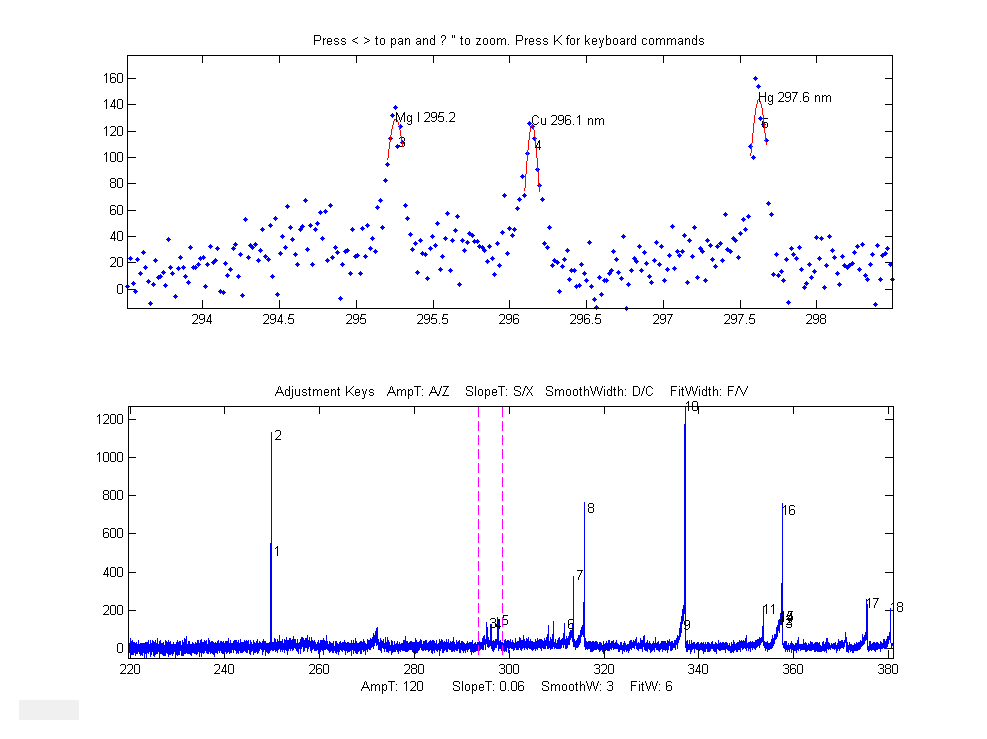Three peaks near 296 nm isolated and identified. Press the I key to display the peak ID names.

###Pressing "O" prints the peak positions, names, errors, and amplitudes in a table in the command window.
Name            Position    Error        Amplitude
'Mg I 295.2'     [295.2]    [0.058545]    [129.27]
'Cu 296.1 nm'    [296.1]    [0.045368]    [124.6]
'Hg 297.6 nm'    [297.6]    [0.023142]    [143.95]

Here is another example, from a large atomic emission spectrum with over 10,000 data points and many hundreds of peaks. The reference table of known peaks in this case is taken from Table 1 of  ASTM C1301 - 95(2009)e1. With the settings I was using, 10 peaks were identified, shown in the table below. You can see that some of these elements have more than one line identified. Obviously, the lower the settings of the AmpThreshold, SlopeThreshold, and SmoothWidth, the more peaks will be detected; and the higher the setting of "MaxError", the more peaks will be close enough to be considered identified. In this example, the element names in the table below are hot-linked to the screen image of the corresponding peak detected as identified by iPeak. Some of these lines, especially Nickel 231.66nm, Silicon 288.18nm, and Iron 260.1nm, are rather weak and have poor signal-to-noise ratios, so their identification might be in doubt (especially Iron, since its wavelength error is greater than the rest). It's up to the experimenter to decide which peaks are strong enough to be significant. In this example, I used an independently published table of element wavelengths, rather than data acquired on that same instrument, so I am depending on the accurate wavelength calibration of the instrument. The evidence suggests that the wavelength calibration is excellent, based on the very small error for the two well-known and relatively strong Sodium lines at 589 and 589.59 nm.  (Even so, I set MaxError to 0.2 nm in this example to loosen up the wavelength matching requirements).

'Name'        'Position'     'Error'      'Amplitude'
'Cadmium'     [  226.46]   [-0.039471]   [   44.603]
'Nickel'      [  231.66]   [ 0.055051]   [   26.381]
'Silicon'     [  251.65]   [ 0.041616]   [   45.275]
'Iron'        [   260.1]   [    0.156]   [    38.04]
'Silicon'     [  288.18]   [ 0.022458]   [   27.214]
'Strontium'   [  421.48]   [-0.068412]   [   41.119]
'Barium'      [  493.35]   [-0.057923]   [   72.466]
'Sodium'      [     589]   [0.0057964]   [   405.23]
'Sodium'      [  589.57]   [-0.015091]   [    315.2]
'Potassium'   [  766.54]   [ 0.051585]   [   61.987]

Note: The ZIP file contains the latest version of the iPeak function as well as some sample data to demonstrate peak identification (Example 8).  Obviously for your own applications, it's up to you to provide your own array of known peak maximum positions ('Positions') and matching cell array of names ('Names') for your particular types of signals.

Keyboard Controls (version 8):
Pan signal left and right...Coarse pan: < or >
Fine pan: left or right cursor arrow keys

Nudge
one point left or right: [ and ]

Zoom in and out.............Coarse zoom: / or '
Fine zoom: up or down cursor arrow keys
Resets pan and zoom.........ESC

Select entire signal........Ctrl-A
Refresh entire plot.........Enter (Updates cursor position in lower plot)
Change plot color...........Shift-C (cycles through standard colors)
Adjust AmpThreshold.........A,Z  (Larger values ignore short peaks)
Type in AmpThreshold........Shift-A  (Type value and press Enter)
Type in SlopeThreshold......Shift-S  (Type value and press Enter)
Adjust SmoothWidth..........D,C  (Larger values ignore sharp peaks}
Toggle sharpen mode ........H  Helps detect overlapped peaks.
Enter de-tailing factor.....Shift-Y.  Removes exponential peak tails

Baseline correction.........B, then click baseline at multiple points
Restore original signal.....G  to cancel previous background subtraction
Invert signal...............-  Invert (negate) the signal (flip + and -)
Set minimum to zero.........0 (Zero) Sets minimum signal to zero

Interpolate signal..........Shift-I  Interpolate (re-sample) to N points
Toggle log y mode OFF/ON....Y
Plot log Y axis on lower graph
Cycles baseline modes.......T 0=none; 1=linear; 2=quad; 3=flat;
4=linear mode(y); 5=flat mode(y)
Toggle valley mode OFF/ON...U  Switch to valley mode
Gaussian/Lorentzian switch..Shift-G
Cycle between Gaussian, Lorentzian,
and flat-top modes
Print peak table............P  Prints Peak #, Position, Height, Width
Save peak table.............Shift-P  Saves peak table as disc file
Step through peaks..........Space/Tab  Jumps to next/previous peak

Normal peak fit.............N  Fit peaks in upper window with peakfit.m
Multiple peak fit...........M  Fit all peaks in signal with peakfit.m
Ensemble average all peaks..Shift-E  (Read instructions first)
MeasUre peak areas .........Shift-U   by perp. drop and Tan. skim.
Print keyboard commands.....K  Prints this list
Print findpeaks arguments...Q  Prints findpeaks function with arguments.
Print ipeak arguments.......W  Prints ipeak function with all arguments.
Print report................R  Prints Peak table and parameters
Print peak statistics.......E  prints mean, std of peak intervals, heights, etc.
Peak labels ON/OFF......... L  Label all peaks detected in upper window.
Peak ID ON/OFF..............I  Identifies closest peaks in
'Names' database.
Print peak IDs..............O  Prints table of peaks IDs

Switch to ipf.m.............Shift-Ctrl-F  Transfer current signal to

Interactive Peak Fitter

Switch to iSignal...........Shift-Ctrl-S  Transfer current signal
to iSignal.m

###demoipeak.m is a simple demo function that generates a noisy signal with peaks, calls iPeak, and then prints out a table of the actual peak parameters and a list of the peaks detected and measured by iPeak for comparison. Before running this demo, ipeak.m must be downloaded and placed in the Matlab path. The ZIP file at http://terpconnect.umd.edu/~toh/spectrum/ipeak7.zip contains several demo functions (ipeakdemo.m, ipeakdemo1.m, etc) that illustrate various aspects of the iPeak function and how it can be used effectively. Download the zip file, right-click and select "Extract all", then put the resulting files in the Matlab path and run them by typing their names at the Matlab command window prompt. To test for the proper installation and operation of iPeak, run testipeak.

 ipeakdemo: effect of the peak detection parameters   Four Gaussian peaks with the same heights but different widths (10, 30, 50 and 70 units) This demonstrates the effect of SlopeThreshold and SmoothWidth on peak detection. Increasing SlopeThreshold (S key) will discriminate against the broader peaks. Increasing SmoothWidth (D key) will discriminate against the narrower peaks and noise. FitWidth (F/V keys) controls the number of points around the "top part" of the (unsmoothed) peak that are taken to estimate the peak heights, positions, and widths. A reasonable value is ordinarily about equal to 1/2 of the number of data points in the half-width of the peaks. In this case, where the peak widths are quite different, set it to about 1/2 of the number of data points in the narrowest peak.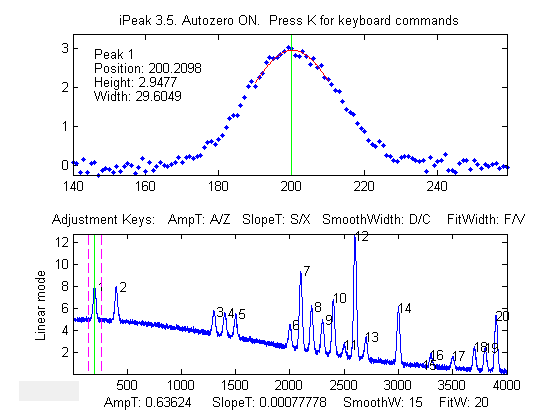ipeakdemo1: the baseline correction mode Demonstration of background correction, for separated, narrow peaks on a large baseline. Each time you run this demo, you will get a different set of peaks and noise. A table of the actual peak positions, heights, widths, and areas is printed out in the command window. Jump to the next/previous peaks using the Spacebar/Tab keys. Hint: Set the linear baseline correction mode (T key), adjust the zoom setting so that the peaks are shown one at a time in the upper window, then press the P key to display the peak table. Try the mode(y) method also.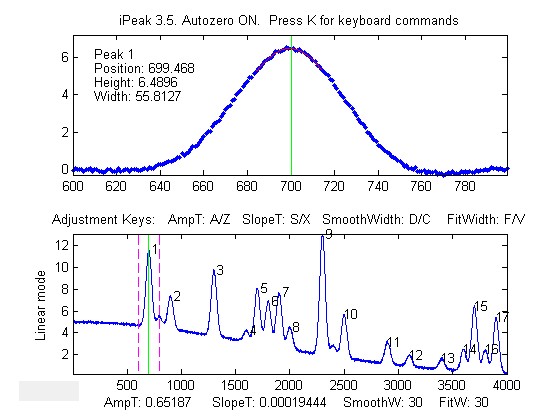ipeakdemo2: peak overlap and the curve fitting functions. Demonstration of error caused by overlapping peaks on a large offset baseline. Each time you run this demo, you will get a different set of peaks and noise. A table of the actual peak positions, heights, widths, and areas is printed out in the command window. (Jump to the next/previous peaks using the Spacebar/Tab keys). Hint: Use the B key and click on the baseline points, then press the P key to display the peak table. Or select the background correction mode (T key) and use the Normal curve fit (N key) with peak shape 1 (Gaussian).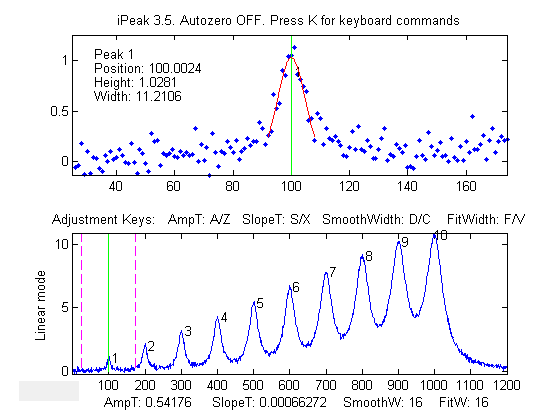ipeakdemo3:  Baseline shift caused by overlapping peaks Demonstration of overlapping Lorentzian peaks, without an added background. A table of the actual peak positions, heights, widths, and areas is printed out in the command window; in this example, the true peak heights are 1,2 3,...10. Overlap of peaks can cause significant errors in measuring peak parameters, especially for Lorentzian peaks, because they have gently sloping sides that contribute to the baseline of any peaks in the region. Hint: turn OFF the background correction mode (T key) and use the Normal curve fit (N key) to fit small groups of 2-5 peaks numbered in the upper window, with peak shape 2 (Lorentzian). For the greatest accuracy in measuring a particular peak, include one or two additional peaks on either side, to help account for the baseline shift caused by the sides of those neighboring peaks. Alternatively, if the total number of peaks is not too great, you can use the Multiple curve fit (M key) to fit the entire signal in the lower window.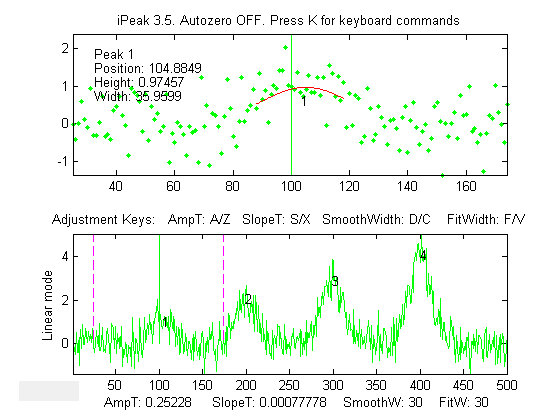ipeakdemo4: dealing with very noisy signals Detection and measurement of four peaks in a very noisy signal. The signal-to-noise ratio of first peak is 2. Each time you run this demo, you will get a different set of noise. A table of the actual peak positions, heights, widths, and areas is printed out in the command window. Jump to the next/previous peaks using the Spacebar/Tab keys. The peak at x=100 is usually detected, but the accuracy of peak parameter measurement is poor because of the low signal-to-noise ratio. ipeakdemo6 is similar but has the option of different kinds on noise (white, pink, proportional, etc). Hint: With very noisy signals it is usually best to increase SmoothWidth and FitWidth to help reduce the effect of the noise.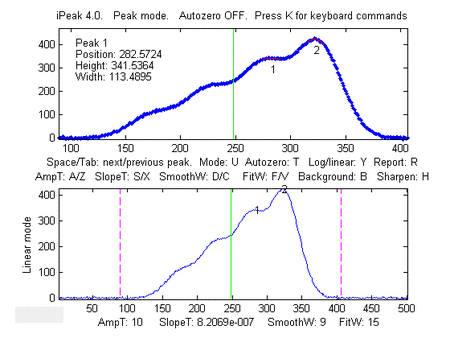ipeakdemo5: dealing with highly overlapped peaks In this demo the peaks are so highly overlapped that only one or two of the highest peaks yield distinct maxima that are detected by iPeak. The height, width, and area estimates are highly inaccurate because of this overlap. The normal peak fit function ('N' key) would be useful in this case, but it depends on iPeak for the number of peaks and for the initial guesses, and so it would fit only the peaks that were found and numbered. To help in this case, pressing the 'H' key will activate the peak sharpen function that decreases peak width and increases peak height of all the peaks, making it easier to detect and number all the peaks for use by the peakfit function (N key). Note: peakfit fits the original unmodified peaks; the sharpening is used only to help locate the peaks to provide peakfit with suitable starting values..

Which to use: iPeak, ipf, or peakfit?  Download these Matlab demos that compare iPeak.m with ipf.m and peakfit.m for signals with a few peaks and signals with many peaks and that shows how to adjust iPeak to detect broad or narrow peaks. These are self-contained demos that include all required Matlab functions. Just place them in your path and click Run or type their name at the command prompt. Or you can download all these demos together in idemos.zip. For peak fitting, the N-key and M-key functions of iPeak have the advantage of using the peak positions and widths determined by the automatic peak finder routine as the first-guess vector for peakfit.m, often resulting in faster and more robust fits that ipf.m or peakfit.m themselves with default first guesses. Try fitting the x,y data in ipeakexampledata.mat for some examples of using iPeak for fitting overlapping peaks. On the other hand, ipf.m is better for fitting peaks that don't make distinct maxima and thus are not detected detected by the automatic peak finder routine.

### Peak Finding and Measurement Spreadsheets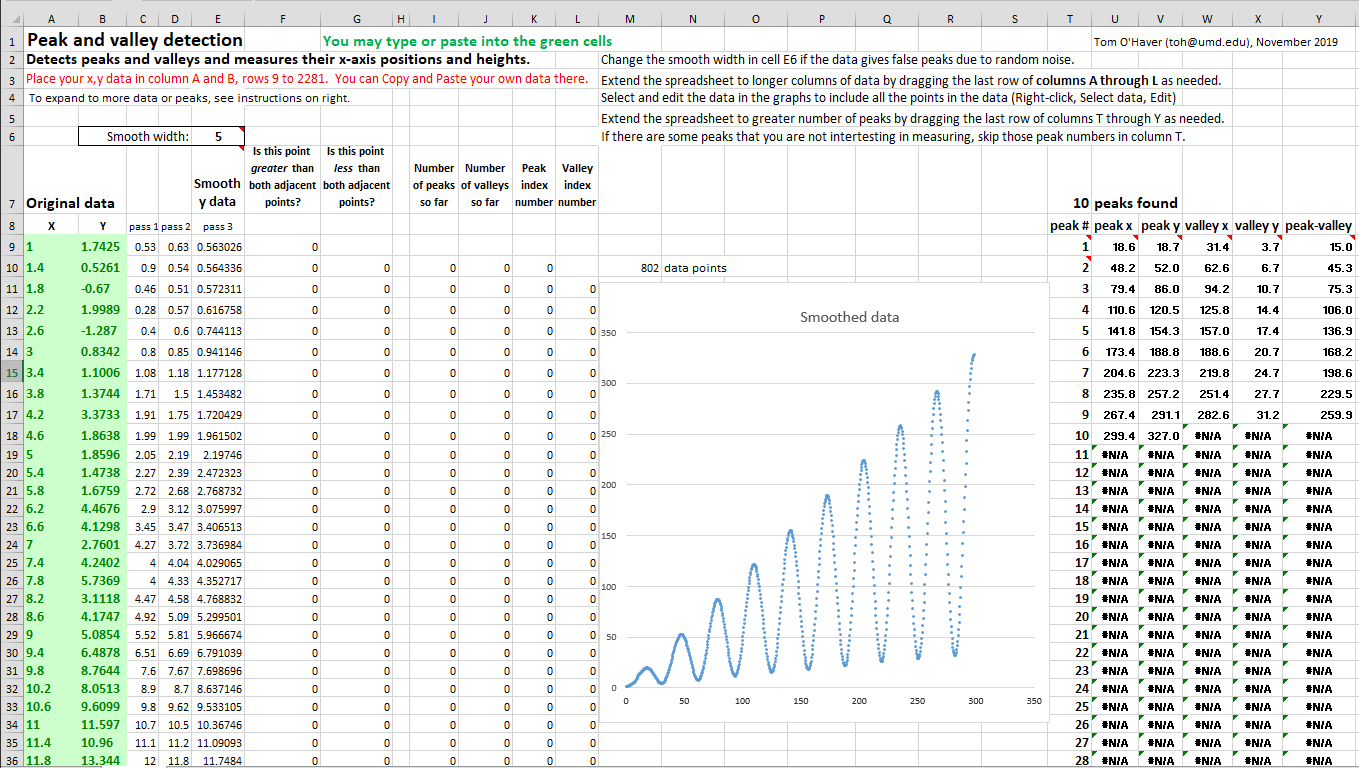Simple peak and valley detection. The spreadsheet pictured above, PeakAndValleyDetectionTemplate.xlsx (or PeakAndValleyDetectionExample.xlsx with sample data), is a simple peak and valley detector that defines a peak as any point with lower points on both sides and a valley as any point with higher points on both sides. Peaks and valleys are marked by colored cells in columns F through L and tabulated in columns T through Y with their measured x and y values based on the use of the INDIRECT, ADDRESS, and MATCH functions (as described by the cell notes on cells T9 to W9). The raw data can be optionally smoothed by entering a smooth width (a positive odd integer) in cell E6 in order to suppress false detection caused by random noise. Simple directions for expanding this template to a larger number of data points or peaks is included.

Selective peak detection. The spreadsheet PeakDetectionTemplate.xls (or PeakDetectionExample.xls with sample data) implements the first-derivative zero-crossing peak detection method with amplitude and slope thresholds as described on page 202. The input x,y data are contained in Sheet1, column A and B, rows 9 to 1200. (You can type or paste your own data there). The amplitude threshold and slope threshold are set in cells B4 and E4, respectively. Smoothing and differentiation are performed by the convolution technique used by the spreadsheets DerivativeSmoothing.xls described previously. The Smooth Width and the Fit Width are both controlled by the number of non-zero convolution coefficients in row 6, columns J through Z. (In order to compute a symmetrical first derivative, the coefficients in columns J to Q must be the negatives of  the positive coefficients in columns S to Z). The original data and the smoothed derivative are shown in the two charts. To detect peaks in the data, a series of three conditions are tested for each data point in columns F, G, and H, corresponding to the three nested loops in findpeaksG.m:

1. Is the signal greater than Amplitude Threshold? (line 45 of findpeaksG.m; column F in the spreadsheet)
2. Is there a downward directed zero crossing in the smoothed first derivative? (line 43 of findpeaksG.m; column G in the spreadsheet)
3. Is the slope of the derivative at that point greater than the Slope Threshold? (line 44 of findpeaksG.m; column H in the spreadsheet)

If the answer to all three questions is yes (highlighted by blue cell coloring), a peak is registered at that point (column I), counted in column J, and assigned an index number in column K. The peak index numbers, X-axis positions, and peak heights are listed in columns AC to AF. Peak heights are computed two ways: "Height" is based on slightly smoothed Y values (more accurate if the peaks are broad and noisy, as in PeakDetectionDemo2b.xls) and "Max" is the highest individual Y value near the peak (more accurate if the data are smooth or if the peaks are very narrow, as in PeakDetectionDemo2a.xls). PeakDetectionDemo2.xls/xlsx is a demonstration with a user-controlled computer-generated series of four noisy Gaussian peaks with known peak parameters. PeakDetectionSineExample.xls is a demo that generates a sinusoidal signal with an adjustable number of peaks.

You can extend the spreadsheets to longer columns of data by dragging the last row of columns A through K as needed, then select and edit the data in the graphs to include all the points in the data (Right-click, Select data, Edit). You can Extend the spreadsheet to greater number of peaks by dragging the last row of columns AC and AD as needed. Edit R7 and the data range in the equations of cells in row 9, columns U, V, WX, AE, and AF to include all the rows containing data, then copy-drag them down to cover all expected peaks.

Peak detection with least-squares measurement. An extension of that method is made in the spreadsheet template PeakDetectionAndMeasurement.xlsx (screen image), which makes the assumption that the peaks are Gaussian and measures their height, position, and width more precisely using a least-squares technique, just like "findpeaksG.m". For the first 10 peaks found, the x,y original unsmoothed data are copied to Sheets 2 through 11, respectively, where that segment of data is subjected to a Gaussian least-squares fit, using the same technique as GaussianLeastSquares.xls. The best-fit Gaussian parameter results are copied back to Sheet1, in the table in columns AH-AK.  (In its present form. the spreadsheet is limited to measuring 10 peaks, although it can detect any number of peaks.  Also it is limited in Smooth Width and Fit Width by the 17-point convolution coefficients).

The spreadsheet is available in OpenOffice (ods) and in Excel (xls) and (xlsx) formats. They are functionally equivalent and differ only in minor cosmetic aspects. There are two example spreadsheets (A, and B) with calculated noisy waveforms that you can modify.  Note: To enter data into the .xls and .xlsx versions, click the "Enable Editing" button in the yellow bar at the top.

If the peaks in the data are too much overlapped, they may not make sufficiently distinct maxima to be detected reliably.  If the noise level is low, the peaks can be sharpened artificially by the technique described previously. This is implemented by PeakDetectionAndMeasurementPS.xlsx and its demo version with example data already entered PeakDetectionAndMeasurementDemoPS.xlsx.

Expanding the PeakDetectionAndMeasurement.xlsx spreadsheet to larger numbers of measured peaks is more difficult. You'll have to drag down row 17, columns AC through AK, and adjust the formulas in those rows for the required number of additional peaks, then copy all of Sheet11 and paste it into a series of new sheets (Sheet12, Sheet13, etc), one for each additional peak, and finally adjust the formulas in columns B and C in each of these additional sheets to refer to the appropriate row in Sheet1. Modify these additional equations in the same pattern as those for peaks 1-10. (In contrast to these spreadsheets, the Matlab/Octave findpeaks functions adapt automatically to any length of data and any number of peaks).

To compare the computation speed of this spreadsheet peak finder to the Matlab/Octave equivalent, we can take as an example the spreadsheet PeakDetectionExample2.xls, or PeakDetectionExample2.ods, which computes and plots a test signal consisting of a noisy sine-squared waveform with 300 data points and then detects and measures 10 peaks in that waveform and displays a table of peak parameters.  This is equivalent to the Matlab/Octave script:

tic
x=1:300;
y(1:99)=randn(size(1:99));
y(100:300)=10.*sin(.16.*x(100:300)).^2. + randn(size(x(100:300)));
P=findpeaksplot(x,y,0.005,5,7,7,3);
disp(P)
drawnow
toc

The table below compares the elapsed times measured on a typical PC desktop computer. The speed advantage of Matlab is clear.

 Method Elapsed time Excel spreadsheet ~ 1 sec Calc spreadsheet ~ 1 sec Matlab script 0.132 sec Octave script 0.85 sec

This is a rather small test; many real-world applications have many more data points and many more peaks, in which the speed advantage of Matlab would be more significant.  Moreover, Matlab would be the method of choice if you have a large number of separate data sets to which you need to apply a peak detection/measurement algorithm completely automatically (See Appendix X: Batch processing).

###